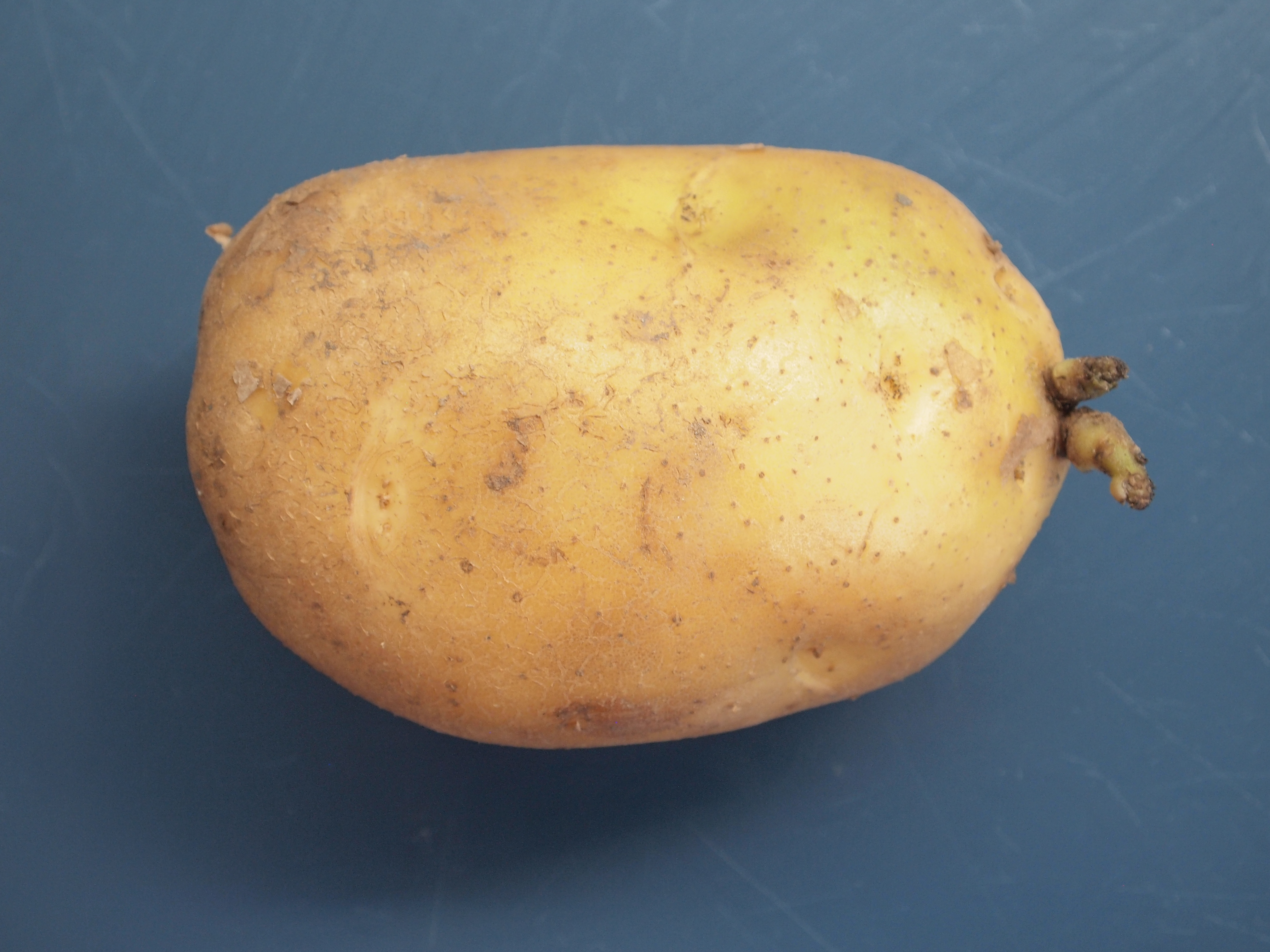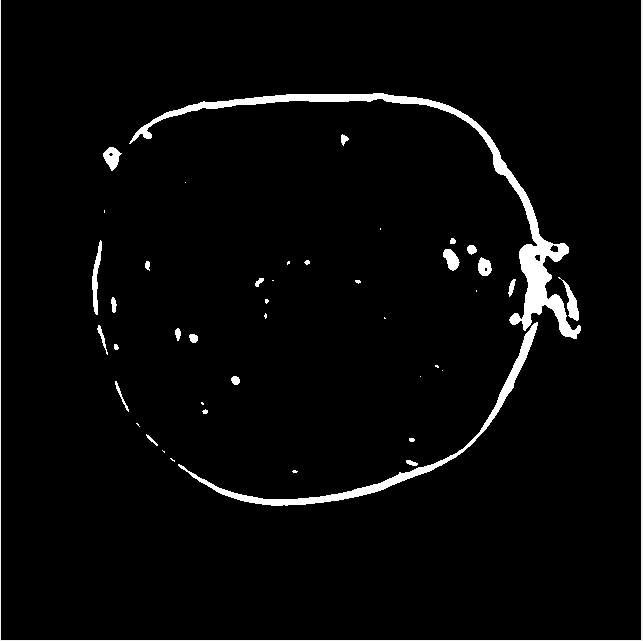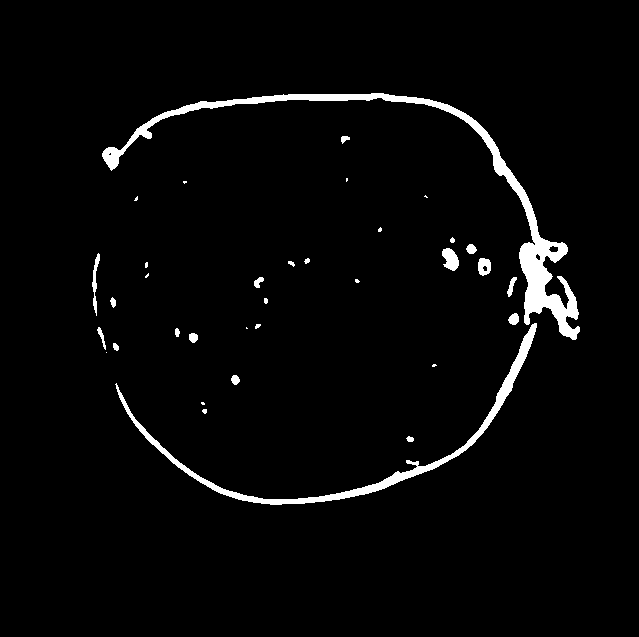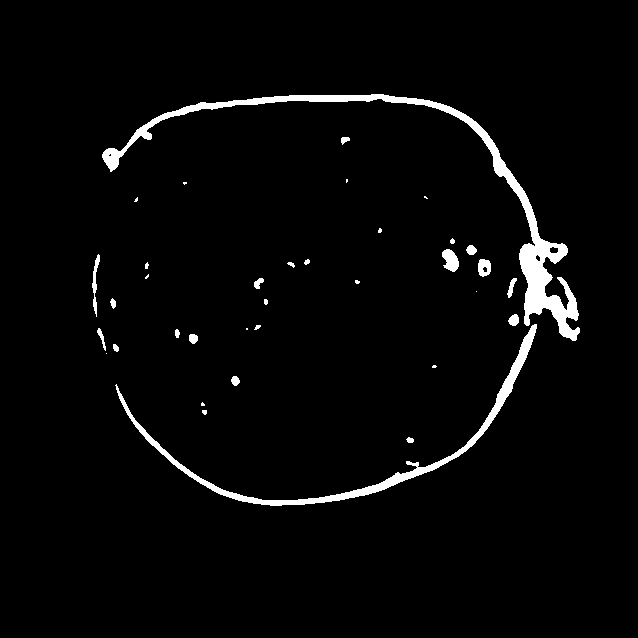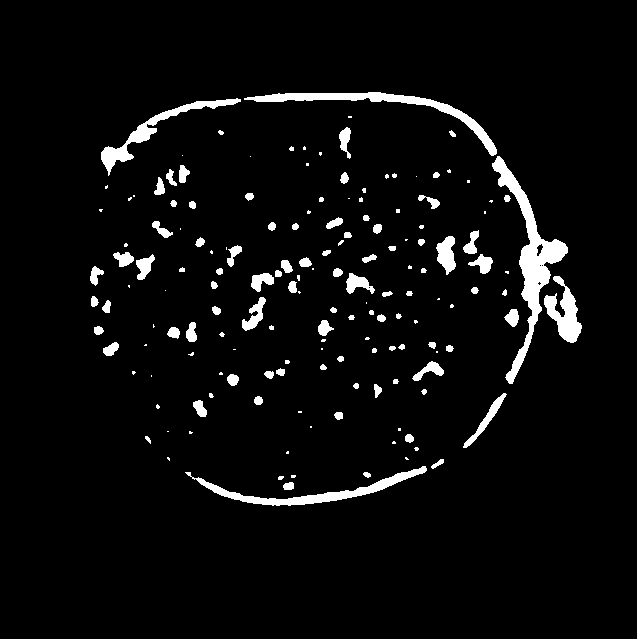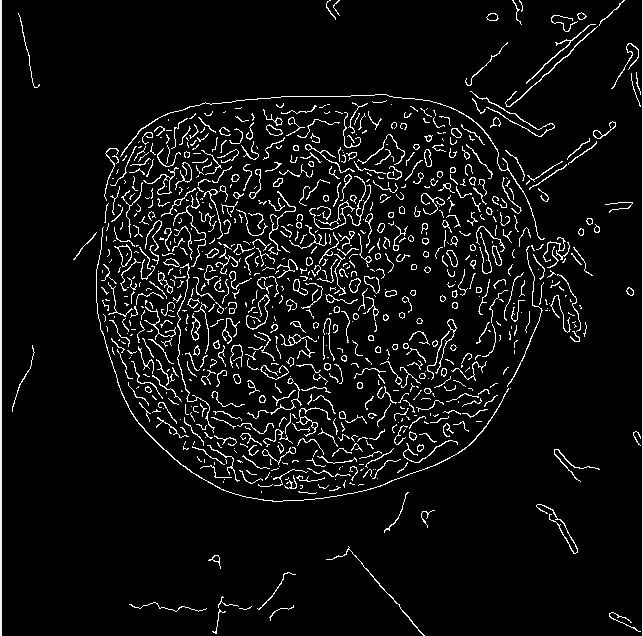# 图像处理边缘分割法

## 数字图像处理｜Matlab-图像分割与边缘检测实验-采用阈值处理方法进行图像分割

2019-12-14 23:09:58 qq_43571150 阅读数 707
• ###### matlab边缘检测和图像分割

matlab边缘检测 <img src="https://img-bss.csdn.net/201903190616489027.jpg" alt="" /></p>

7课时 101分钟 981人学习 刘昱显
免费试看

## Matlab-图像分割与边缘检测实验-采用阈值处理方法进行图像分割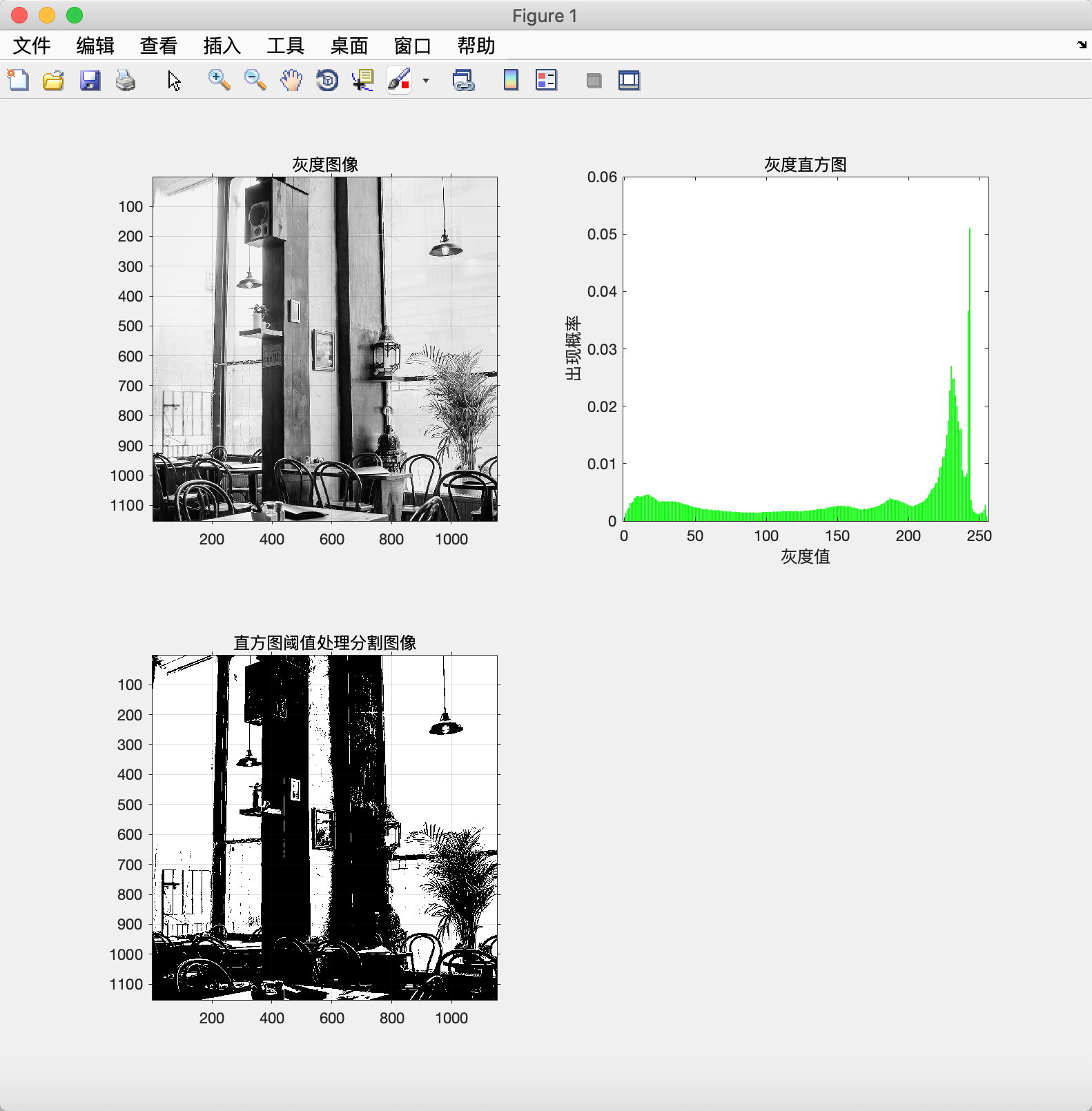Matlab代码👇

I=imread('05.jpg');       %读取当前路径下的图片
I1=rgb2gray(I);
subplot(2,2,1);imshow(I1);title('灰度图像');

grid on;                        %显示网格线
axis on;                        %显示坐标系
[m,n]=size(I1);                 %测量图像尺寸参数
GK=zeros(1,256);                %预创建存放灰度出现概率的向量
for k=0:255
GK(k+1)=length(find(I1==k))/(m*n);             %计算每级灰度出现的概率，将其存入GK中相应位置
end
subplot(2,2,2),bar(0:255,GK,'g')                    %绘制直方图

title('灰度直方图')
xlabel('灰度值')
ylabel('出现概率')
I2=im2bw(I,200/255);
subplot(2,2,3),imshow(I2);title('直方图阈值处理分割图像')
imwrite(I2,'05 直方图阈值200的分割图像.jpg');
grid on;                         %显示网格线
axis on;                         %显示坐标系


## 图像处理之图像分割（二）之边缘分割之边缘松弛

2017-12-21 21:42:15 coming_is_winter 阅读数 1131
• ###### matlab边缘检测和图像分割

matlab边缘检测 <img src="https://img-bss.csdn.net/201903190616489027.jpg" alt="" /></p>

7课时 101分钟 981人学习 刘昱显
免费试看

对于书中的边缘松弛算法进行一下拓展，，便于理解。代码方面还没头绪，后续再说。

## 图像分割·基于边缘检测

2017-10-30 11:20:54 u010189457 阅读数 26833
• ###### matlab边缘检测和图像分割

matlab边缘检测 <img src="https://img-bss.csdn.net/201903190616489027.jpg" alt="" /></p>

7课时 101分钟 981人学习 刘昱显
免费试看

## 一、图像边缘检测

基本思路：基于边缘检测的图像分割方法的基本思路是先确定图像中的边缘像素，然后再把这些像素连接在一起就构成所需的区域边界。

图像边缘：图像边缘，即表示图像中一个区域的终结和另一个区域的开始，图像中相邻区域之间的像素集合构成了图像的边缘。所以，图像边缘可以理解为图像灰度发生空间突变的像素的集合。图像边缘有两个要素，即：方向和幅度。沿着边缘走向的像素值变化比较平缓；而沿着垂直于边缘的走向，像素值则变化得比较大。因此，根据这一变化特点，通常会采用一阶和二阶导数来描述和检测边缘。

综上，图像中的边缘检测可以通过对灰度值求导数来确定，而导数可以通过微分算子计算来实现。在数字图像处理中，通常是利用差分计算来近似代替微分运算。梯度幅值计算

设f(x,y)$f(x,y)$为连续图像函数，Gx$G_x$Gy$G_y$分别为x$x$方向和y$y$方向的梯度，且在点(x,y)$(x,y)$处的梯度可以表示为一个矢量，梯度定义如下：

G(f(x,y))=[f(x,y)x f(x,y)y]T$G(f(x,y))=[\frac{\partial f(x,y)}{\partial x}\ \frac{\partial f(x,y)}{\partial y}]^T$

对应欧式距离梯度幅值：

|G(x,y)|=G2x+G2y$|G(x,y)|=\sqrt {G_x^2+G_y^2}$

对应棋盘距离梯度幅值：

|G4(x,y)|=|Gx|+|Gy|$|G_4(x,y)| =|G_x|+|G_y|$

对应街区距离梯度幅值：

|G8(x,y)|=max{|Gx|+|Gy|}$|G_8(x,y)| = max\{|G_x|+|G_y|\}$梯度矢量幅角表示的梯度方向是函数f(x,y)$f(x,y)$增加最快的方向：

ϕ(x,y)=arctan(Gx/Gy)$\phi (x,y) = arctan(G_x/G_y)$

## 二、微分算子

常用的一阶微分算子有Roberts、Prewitt、Sobel等算子，常用的二阶微分算子有Laplace和Kirsh等算子。在实际处理操作中常用模板矩阵与图像像素值矩阵卷积来实现微分运算。

由于垂直边缘的方向上的像素点和噪声都是灰度不连续点，所以变换到频域时，在频域均为高频分量，直接采用微分运算不可避免地会受到噪声的很大影响。 因此，微分算子只适用于图像噪声比较少的简单图像。针对此问题，LoG算子（Laplace of Gaussian）和Canny算子采取的方法是，先对图像进行平滑滤波，然后再用微分算子与图像进行卷积操作，这样处理会得到比较好的边缘检测结果。其中，LoG算子是采用Laplacian算子计算高斯函数的二阶导数，Canny算子是高斯函数的一阶导数，两种算子在抑制噪声和检测边缘之间取得了比较好的平衡。

### 1. Roberts算子

Robert算子是一种利用局部差分算子检测边缘的算子，对具有陡峭的低噪声图像处理的效果较好。其定义如下:

G[f(x,y)]={[f(x+1,y+1)f(x,y)]2+[f(x+1,y)f(x,y+1)]2}12$G[f(x,y)] = \{[f(x+1,y+1)-f(x,y)]^2+[f(x+1,y)-f(x,y+1)]^2\}^{\frac12}$

上述公式计算量较大，在实际操作中通常采用绝对差算法近似代替上述公式：

G[f(x,y)]|f(x+1,y)f(x,y)|+|f(x,y+1)f(x,y)|$G[f(x,y)] \approx |f(x+1,y)-f(x,y)|+|f(x,y+1)-f(x,y)|$

G[f(x,y)]|f(x+1,y+1)f(x,y)|+|f(x,y+1)f(x+1,y)|$G[f(x,y)] \approx |f(x+1,y+1)-f(x,y)|+|f(x,y+1)-f(x+1,y)|$

Roberts算子卷积核：

$\left[ \begin{matrix} 1 & 0 \\ 0 & -1 \end{matrix} \right]$ $\left[ \begin{matrix} 0 & 1 \\ -1 & 0 \end{matrix} \right]$

Roberts算子概念简单，算法计算复杂度较低，对低噪声图像操作可以得到不错的效果，但是在稍复杂图像上则难以胜任。

### 2. Sobel算子

Sobel算子有两个矩阵算子，也称卷积核，分别计算x$x$方向和y$y$方向上的方向梯度，两个核分别与图像进行卷积计算，一个对水平方向上的边缘敏感，一个对垂直方向上的边缘敏感。Sobel算子定义如下：

S=(d2x+d2y)12$S = (d_x^2+d_y^2)^\frac 12$

dx=[f(x1,y1)+2f(x,y1)+f(x+1,y1)][f(x1,y+1)+2f(x,y+1)+f(x+1,y+1)]$d_x = [f(x-1,y-1)+2f(x,y-1)+f(x+1,y-1)]-[f(x-1,y+1)+2f(x,y+1)+f(x+1,y+1)]$

dy=[f(x+1,y1)+2f(x+1,y)+f(x+1,y+1)][f(x1,y1)+2f(x1,y)+f(x1,y+1)]$d_y = [f(x+1,y-1)+2f(x+1,y)+f(x+1,y+1)]-[f(x-1,y-1)+2f(x-1,y)+f(x-1,y+1)]$

用模板表示dx$d_x$dy$d_y$如下：

101202101$\left[ \begin{matrix} -1 & -2 & -1 \\ 0 & 0 & 0 \\ 1 & 2 &1 \end{matrix} \right]$ 121000121$\left[ \begin{matrix} -1 & 0 & 1 \\ -2 & 0 & 2 \\ -1 & 0 & 1 \end{matrix} \right]$

Sobel算子结构简洁，能较好地抑制噪声。

### 3. Prewitt算子

Prewitt算子和Sobel算子类似，也有两个卷积核，区别在于卷积核中心系数的权值不同。Prewitt算子定义：
Sp=(d2x+d2y)12$S_p = (d_x^2+d_y^2)^\frac 12$

101101101$\left[ \begin{matrix} -1 & -1 & -1 \\ 0 & 0 & 0 \\ 1 & 1 &1 \end{matrix} \right]$ 111000111$\left[ \begin{matrix} -1 & 0 & 1 \\ -1 & 0 & 1 \\ -1 & 0 & 1 \end{matrix} \right]$

Prewitt算子实现起来比Sobel算子更为简单，也可以一定程度上抑制噪声。

### 4. LoG算子

LoG(Laplace of Gaussian)，即拉普拉斯高斯算子。拉普拉斯算子是对二维函数求二阶导数的算子(f(x,y)2/x2+f(x,y)2/y2)$(\partial f(x,y)^2/\partial x^2+\partial f(x,y)^2/\partial y^2)$，拉普拉斯高斯算子则是对二维高斯函数求二阶导数的算子。
二维高斯函数：

G(x,y)=ex2+y22σ2$G(x,y) = e^{\frac {x^2+y^2}{2\sigma ^2}}$

LoG算子:

ΔG(x,y)=[x2+y22σ2σ4]ex2+y22σ2$\Delta G(x,y) = [\frac {x^2+y^2-2\sigma ^2}{\sigma ^4}]e^{-\frac {x^2+y^2}{2\sigma ^2}}$

LoG卷积核：

010141010$\left[ \begin{matrix} 0 & -1 & 0 \\ -1 & 4 & -1 \\ 0 & -1 & 0 \end{matrix} \right]$111181111$\left[ \begin{matrix} -1 & -1 & -1 \\ -1 & 8 & -1 \\ -1 & -1 & -1 \end{matrix} \right]$

LoG算子可以根据需要进行“调整”以便在期望的尺寸上起作用（比如下面的5x5的卷积核），可使得大的算子可用于检测模糊边缘，小的算子则可以用于检测锐度集中的精细细节。

00100012101216210121000100$\left[ \begin{matrix} 0 & 0 & -1 & 0 & 0 \\ 0 & -1 & -2 & -1 & 0 \\ -1 & -2 & 16 & -2 & -1 \\ 0 & -1 & -2 & -1 & 0 \\ 0 & 0 & -1 & 0 & 0 \end{matrix} \right]$24442408044824844080424442$\left[ \begin{matrix} -2 & -4 & -4 & -4 & -2 \\ -4 & 0 & 8 & 0 & -4 \\ -4 & 8 & 24 & 8 & -4 \\ -4 & 0 & 8 & 0 & -4 \\ -2 & -4 & -4 & -4 & -2 \end{matrix} \right]$

5x5卷积核

LoG算子的特点：
1. 图像与高斯滤波器进行卷积，既平滑了图像又降低了噪声，孤立的噪声点和较小的结构组织将被滤除。
2. 在边缘检测时则仅考虑那些具有局部梯度最大值的点为边缘点，用拉普拉斯算子将边缘点转换成零交叉点，通过零交叉点的检测来实现边缘检测。
3. 缺点是在过滤噪声的同时使得原有的边缘一定程度上被平滑了。

### 5. Canny算子

Canny算子检测边缘点的方法基本思想是寻找图像梯度的局部最大值。
评价一个边缘检测算子的，一般考虑如下三个指标：
1. 低失误概率：在尽可能把所有边缘检测到的同时，减少将非边缘误判为边缘；
2. 高位置精度：检测出的边缘是真正的边界，检测到的边缘位置足够精确；
3. 检测得到的边界是单像素宽。
针对这三个指标，Canny在设计检测算子时提出了边缘检测算子的三个准则：
1. 信噪比准则；
2. 定义精度准则；
3. 单边缘响应准则。

遵循这三个准则，Canny算子设计实现的步骤如下：
（1）首先用高斯滤波模板进行卷积以平滑图像；
（2）利用微分算子，计算梯度的幅值和方向；
（3）对梯度幅值进行非极大值抑制。即遍历图像，若某个像素的灰度值与其梯度方向上前后两个像素的灰度值相比不是最大，那么这个像素值置为0，即不是边缘；
（4）使用双阈值算法检测和连接边缘。即使用累计直方图计算两个阈值，凡是大于高阈值的一定是边缘；凡是小于低阈值的一定不是边缘。如果检测结果大于低阈值但又小于高阈值，那就要看这个像素的邻接像素中有没有超过高阈值的边缘像素，如果有，则该像素就是边缘，否则就不是边缘。
边缘连接：上述方法仅得到处在边缘上的像素点。噪声和不均匀的照明而产生的边缘间断的影响，使得经过边缘检测后得到的边缘像素点很少能完整地描绘实际的一条边缘。可以在使用边缘检测算法后，紧接着使用连接方法将边缘像素组合成有意义的边缘。

## 十六、数字图像处理之图像分割

2019-05-13 09:13:18 mary_0830 阅读数 10847
• ###### matlab边缘检测和图像分割

matlab边缘检测 <img src="https://img-bss.csdn.net/201903190616489027.jpg" alt="" /></p>

7课时 101分钟 981人学习 刘昱显
免费试看

# （一）点、线和边缘检测

## （1）点检测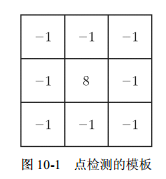g = abs(imfilter(tofloat(f),w))>=T;  %g是包含检测点的图像；f是输入图像；w是点检测模板


f = imread('C:\Users\Public\Pictures\Sample Pictures\Fig0903(a).tif');
subplot(121),imshow(f);
w = [-1 -1 -1;-1 8 -1;-1 -1 -1];
g = abs(imfilter(tofloat(f),w));
T = max(g(:));  %在滤波后图像中选择的最大值
g = g >=T;
subplot(122),imshow(g);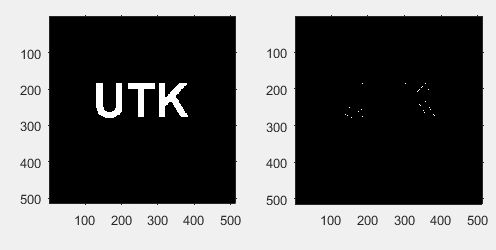g = ordfilt2(f,m*n,ones(m,n))-ordfilt2(f,1,ones(m,n));
g = g>=T;


## （2）线检测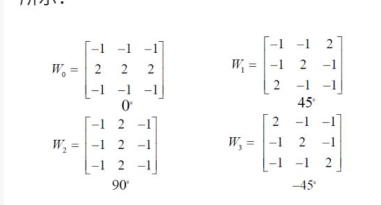f = imread('C:\Users\Public\Pictures\Sample Pictures\Fig0513(a).tif');
subplot(231),imshow(f),title('原始图像');
w = [2 -1 -1;-1 2 -1;-1 -1 2];
g = imfilter(tofloat(f),w);
subplot(232),imshow(g,[]),title('45°处理后的图像');
gtop = g(1:120,1:120);
gtop = pixeldup(gtop,4);
subplot(233),imshow(gtop,[]),title('左上角放大的图像');
gbot = g(end -119:end,end -119:end);
gbot = pixeldup(gbot,4);
subplot(234),imshow(gbot,[]),title('右下角放大的图像');
g =abs(g);
subplot(235),imshow(g,[]),title('绝对值的图像');
T =max(g(:));
g =g >=T;
subplot(236),imshow(g),title('所有点满足g>=T的图像');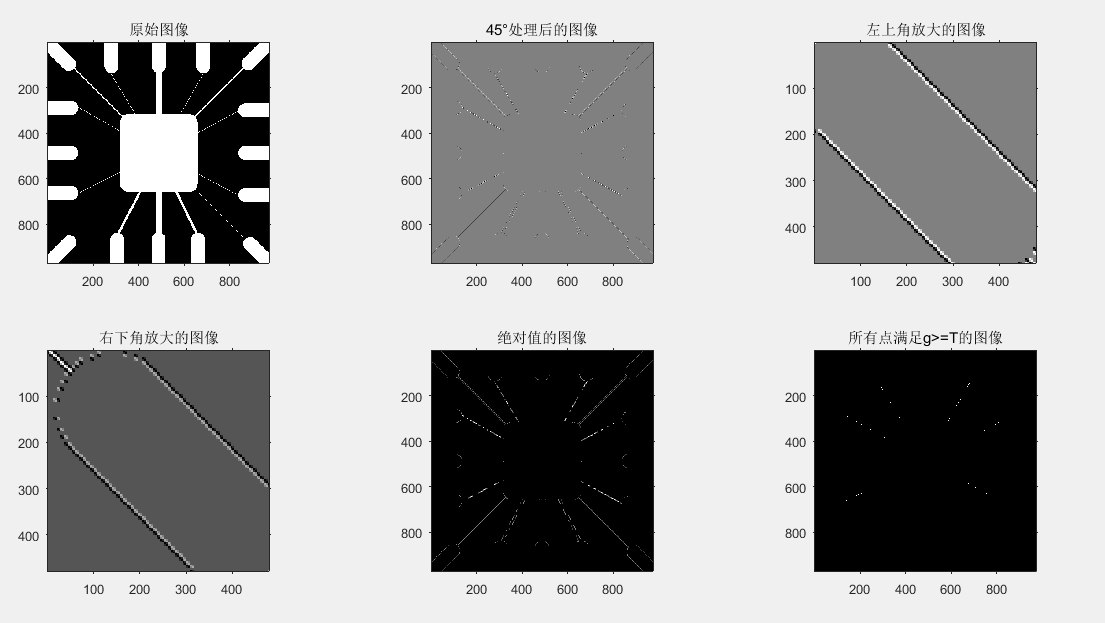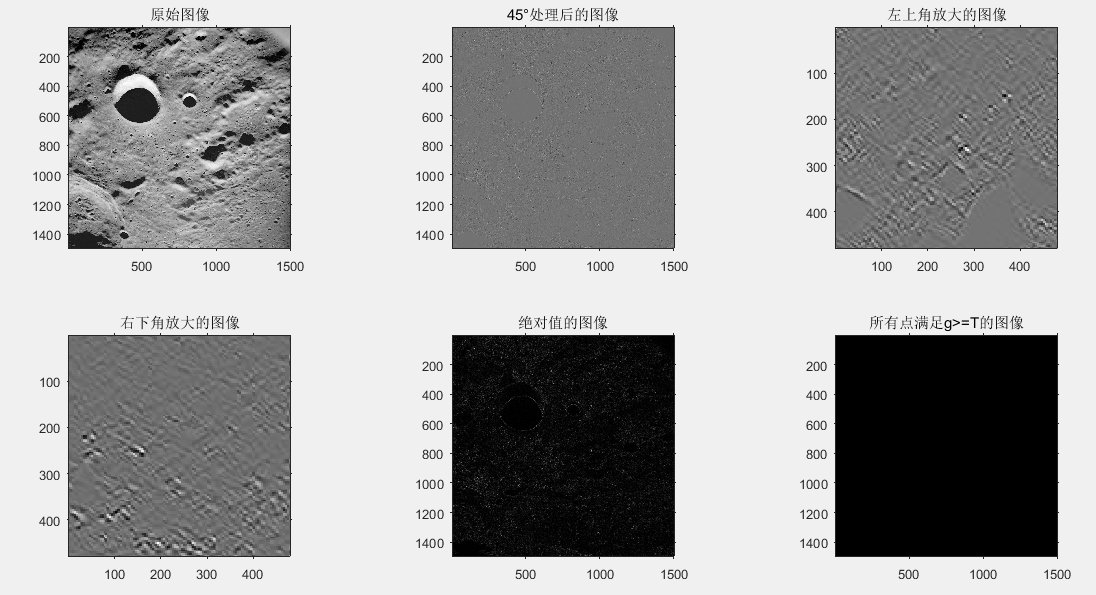实验分析： 在进行45°的边缘检测中，只能够检测到45°或者135°的边缘，并在matlab中进行显示，发现在第二幅图中，45°的边缘检测并不是清楚的看出45°的边缘，而只能看到由点组成的边缘，这是因为原图像中基本没有呈对角线的线条，因此并不能显示对角线的边缘。绝对值的图像是将图像变成了二值图像（只有黑与白），比灰度图像更加清晰看出检测到的线条边缘。

## （3）使用函数edge的边缘检测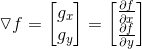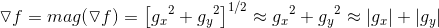[g,t] = edge(f,'method',parameters)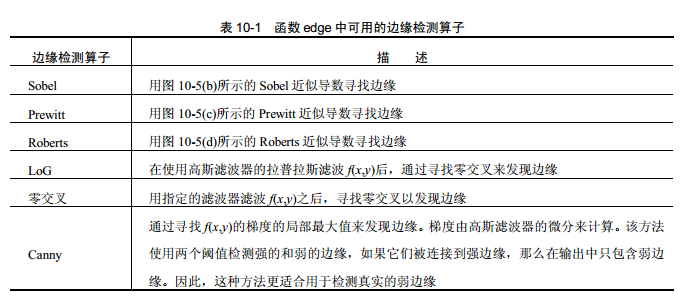下面分别介绍表格中的边缘检测算子：

1. sobel边缘检测算子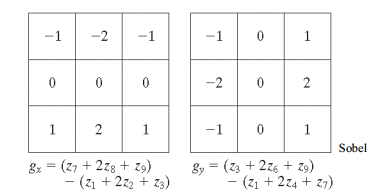调用语法是：
[g,t] = edge(f,'sobel',T,dir)  %T是阈值，dir是边缘检测首选方向：'horizontal'、'vertical'、‘both’

1. prewitt边缘检测算子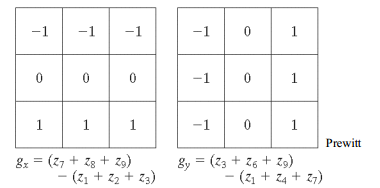调用语法为：（较容易产生噪声）
[g,t] = edge(f,'prewitt',T,dir)  %T是阈值，dir是边缘检测首选方向：'horizontal'、'vertical'、‘both’

1. Roberts边缘检测算子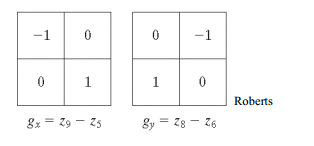调用语法为：
[g,t] = edge(f,'roberts',T,dir)  %T是阈值，dir是边缘检测首选方向：'horizontal'、'vertical'、‘both’

1. LoG检测算子
高斯函数：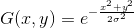这个公式表示的是平滑函数。如果此函数和图像进行卷积，则图像会变得模糊，且模糊的程度是由σ决定的。这个函数的拉普拉斯算法是：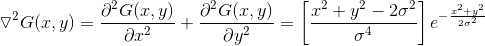这个函数称为LoG算子。原理是：由于求二阶导数是线性操作，所以用这个函数卷积这幅图像与先用平滑函数对图像卷积，再对结果进行拉普拉斯计算的结果是一样的。

[g,t] = edge(f,'log',T,sigma)  %T是阈值，sigma是标准差，默认为2

1. 零交叉检测算子
这种算子与LoG方法类似，不同之处在于卷积使用特殊的滤波函数H来完成的。
调用语法为：
[g,t] = edge(f,'zerocross',T,H)  %T是阈值

1. Canny检测算子
这种算子是edge函数中最强的边缘检测算子
（1）图像用指定了标准差σ的高斯滤波器来平滑，用来减少噪声；
（2）局部梯度和边缘方向在每个点都进行计算。边缘点被定义为梯度方向上局部强度最大的点。
（3）在（2）中决定的边缘点在梯度幅度图像上给出脊。然后算法追踪所有脊的顶部，设置所有的不在脊的顶部的像素为零。因此在输出中给出一条细线，这是非最大值抑制处理。脊像素使用称为“滞后阈值”的技术进行阈值处理，这种技术以使用两个阈值为基础，即T1和T2，且T1<T2。值大于T2的脊像素称为强边缘像素，T1和T2之间的脊像素称为弱边缘像素。
（4）算法用合并8连接的弱像素点到强像素点的方法执行边缘连接。
调用语法为：
[g,t] = edge(f,'canny',T,sigma)  %T是向量=[T1,T2]；sigma是标准差，默认为1


f = imread('C:\Users\Public\Pictures\Sample Pictures\Fig0309(a).tif');
subplot(231),imshow(f),title('原始图像');
[gv,t] = edge(f,'sobel','vertical');
subplot(232),imshow(gv),title('默认阈值垂直的sobel图像');
t
gv = edge(f,'sobel',0.15,'vertical');
subplot(233),imshow(gv),title('阈值0.15垂直的sobel图像')
gboth = edge(f,'sobel',0.15);
subplot(234),imshow(gboth),title('阈值为0.15垂直水平的sobel图像')
wneg45 = [-2 -1 0;-1 0 1;0 1 2];
gneg45 = imfilter(tofloat(f),wneg45,'replicate');
T =0.3*max(abs(gneg45(:)));
gneg45 = gneg45>=T;
subplot(235),imshow(gneg45),title('阈值45°边缘图像')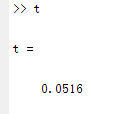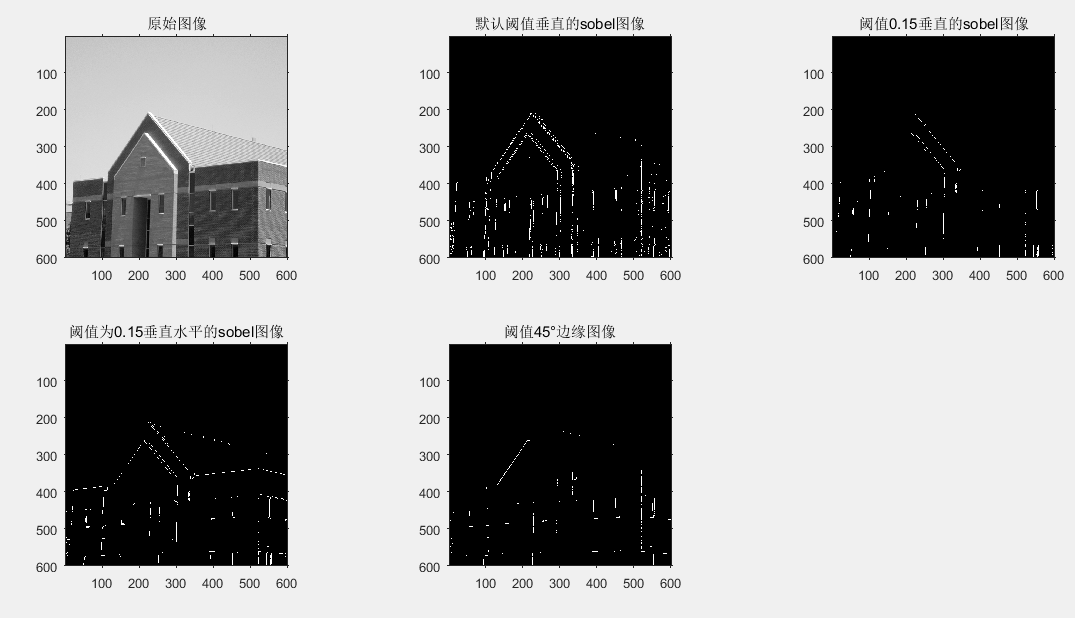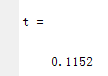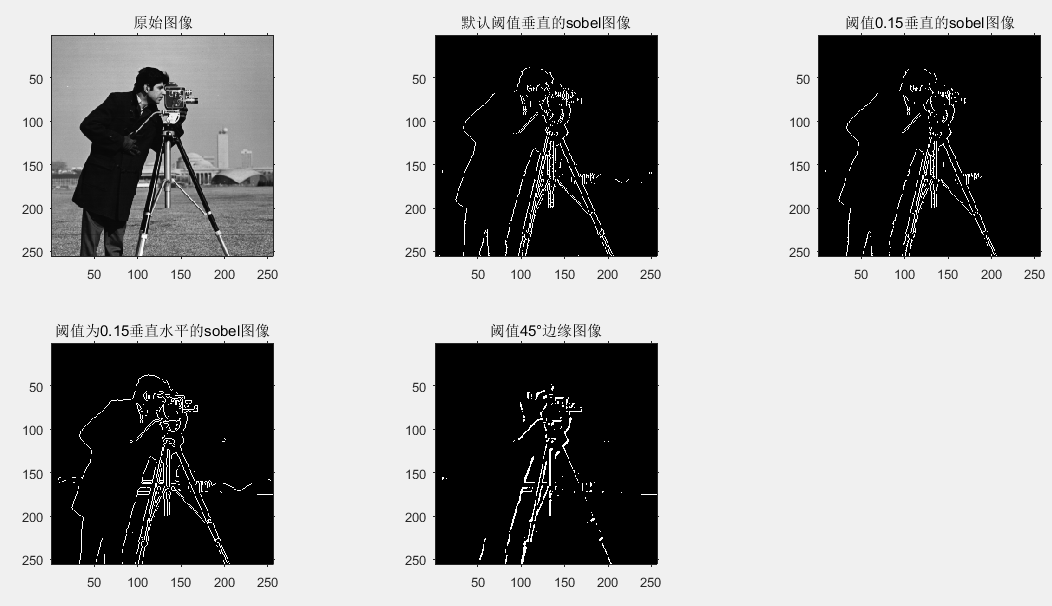实验分析： 当参数是默认垂直的sobel边缘检测时，得到边缘细节是垂直的，水平的边缘线是没有能够检测出来的。当加入参数0.15之后，之前能够检测到的细节却不能够检测出来了，是因为这已经超出了阈值的范围；当采用垂直水平的边缘检测，将之前没有能够检测出的水平线能够检测并显示出来了；当所检测的边缘线是45°的时候，该算法只能够检测到45°或135°的边缘线条，并显示出来。

sobel、Log和canny边缘检测算子进行比较，编写实验代码：

f = tofloat(f);
subplot(221),imshow(f),title('原始图像')
[gSobel_dafault,ts] = edge(f,'sobel');
[gLog_dafault,tlog] = edge(f,'log');
[gCanny_dafault,tc] = edge(f,'canny');
subplot(222),imshow(gSobel_dafault),title('默认的sobel图像');
subplot(223),imshow(gLog_dafault),title('默认的log图像');
subplot(224),imshow(gCanny_dafault),title('默认的canny图像');

gSobel_best = edge(f,'sobel',0.05);
gLog_best = edge(f,'log',0.003,2.25);
gCanny_best = edge(f,'canny',[0.04 0.1],1.5);
subplot(222),imshow(gSobel_best),title('最好效果的sobel图像');
subplot(223),imshow(gLog_best),title('最好效果的log图像');
subplot(224),imshow(gCanny_best),title('最好效果的canny图像');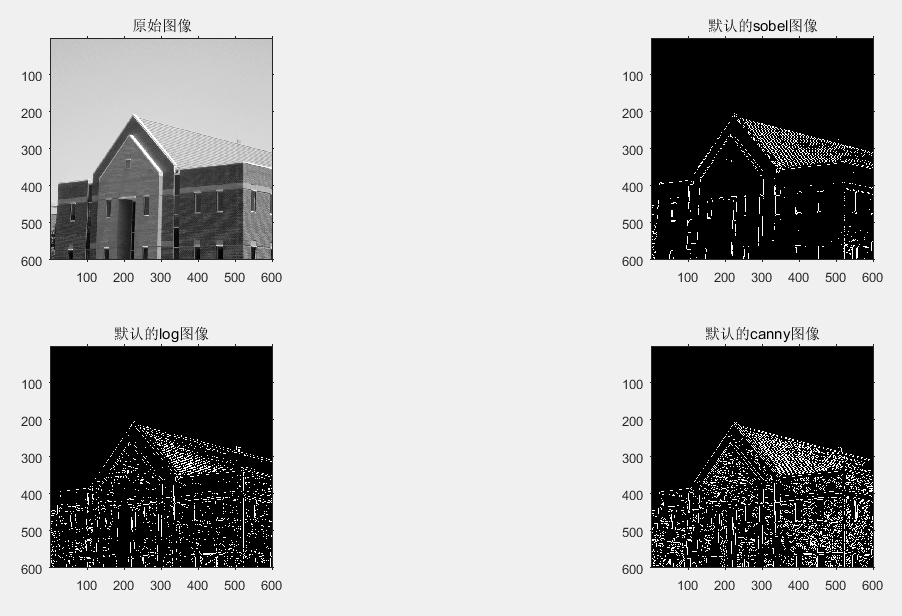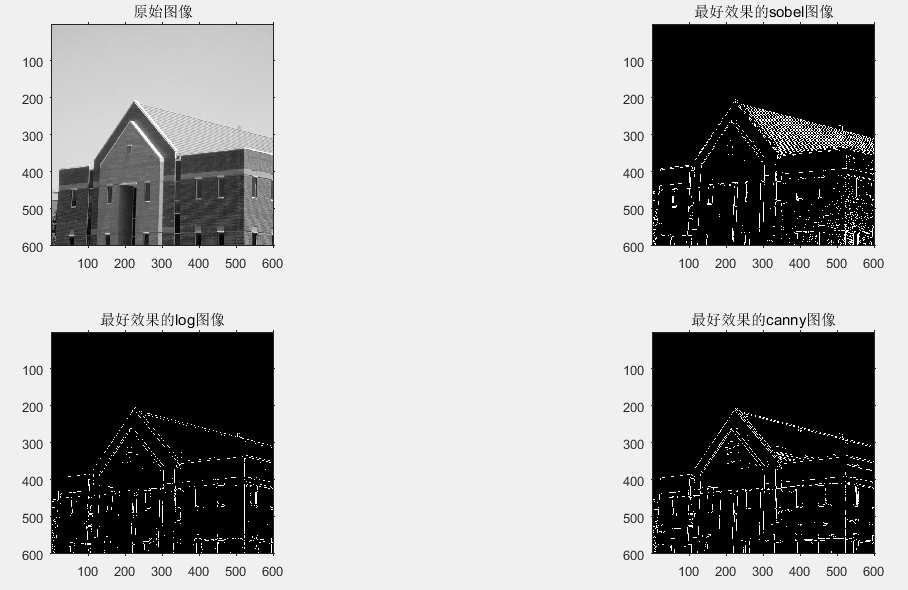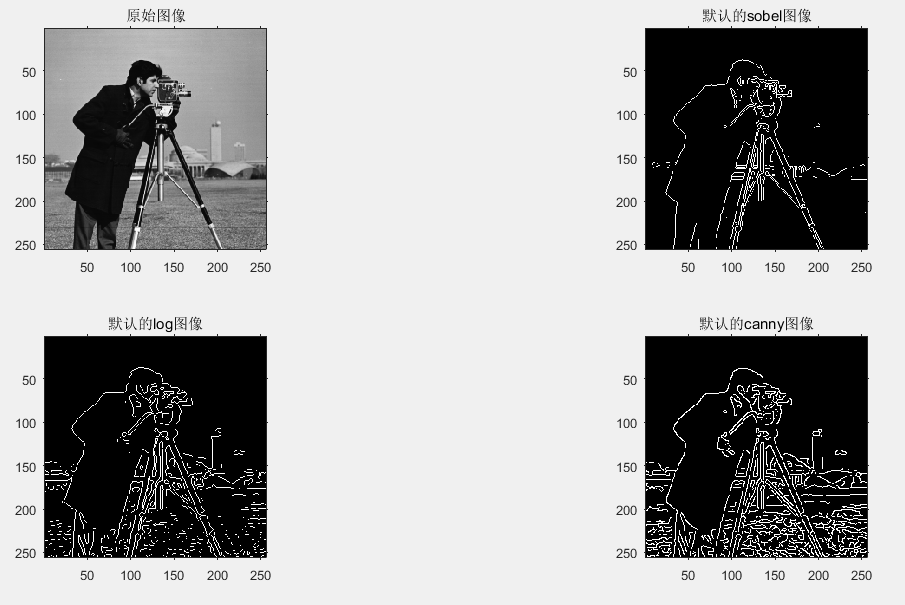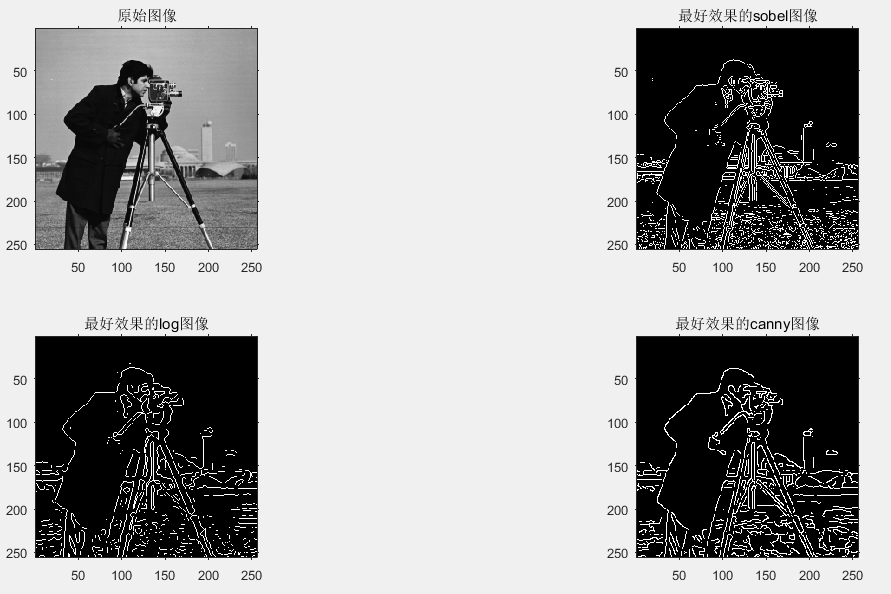实验分析： 同样的一幅图像，使用默认的sobel边缘检测得到的图像中线条是比较其他两种是比较少的，而且获取的边缘细节不是很完整，缺失了很多重要的细节，比如说草地上的细节等。但是在最佳的sobel边缘检测中，所得到的图像是比较完整的，线条细节基本上都被获取并显示出来，相比较其他两种方法，所得到的边缘细节是最完整的。在canny边缘检测中，默认参数的canny检测是最好的，所获得细节是最多的，其边缘基本都可以完整的检测到。

# （二）使用霍夫变换的线检测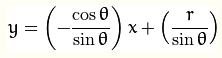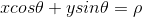1. 函数hough
调用函数：
 [H,theta,rho] = hough(f)
或 [H,theta,rho] = hough(f,'ThetaRes',val1,'RhoRes',val2)


f = zeros(101,101);
f(1,1) = 1;f(101,1) = 1;f(1,101) = 1;
f(101,101) = 1;f(51,51) = 1;
H = hough(f)
imshow(H,[])

[H,theta,rho] = hough(f);
imshow(H,[],'XData',theta,'YData',rho,'InitialMagnification','fit')
axis on,axis normal
xlabel('\theta'),ylabel('\rho')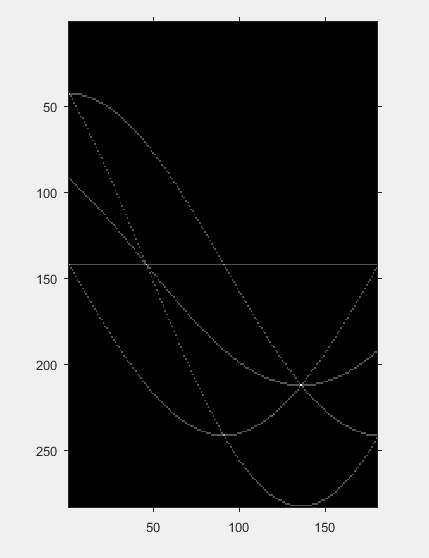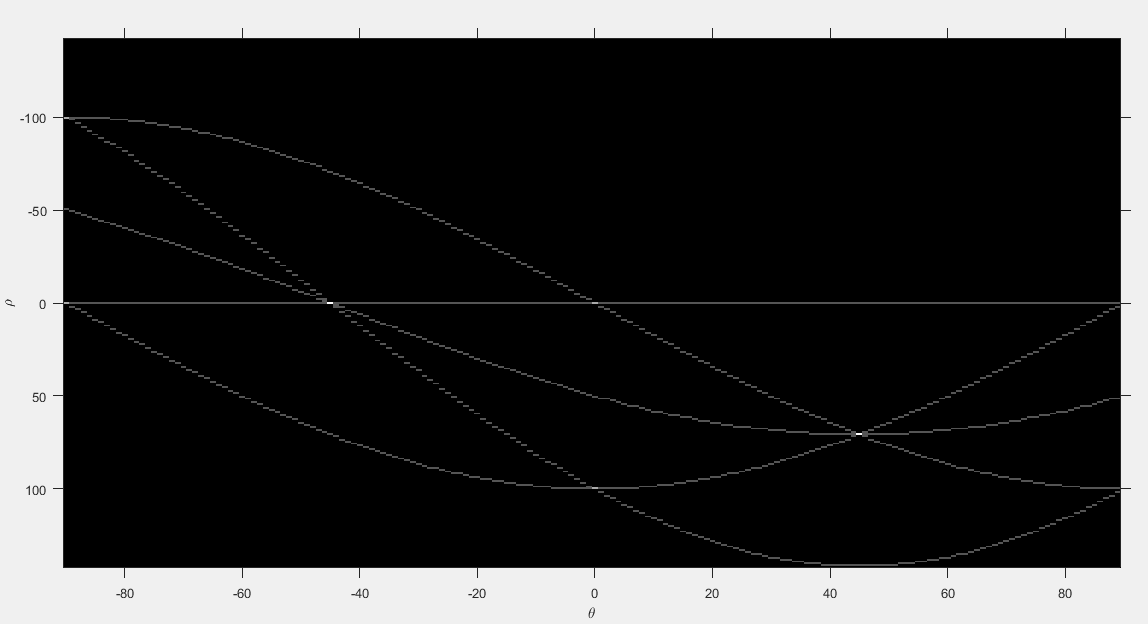实验分析： 三条曲线（直线也可以考虑为曲线）在±45°处的交点指出：f中有两组三个共线的点。两条曲线在(ρ,θ)=(0,-90)、(-100,-90)、(0,0)和(100,0)处的交点指出：有4组位于垂直线和水平线上的共线点。（需要将图像放大以方便观察其坐标）

1. 函数houghpeaks：寻找霍夫变换的峰值（累加单元的高计数）

peaks = houghpeaks(H,NumPeaks)



1. 函数houghlines：当峰值在霍夫变换中被识别出来，则可用此函数来决定线的起始点和终点。
调用函数：
lines = houghlines(f,theta,rho,peaks)



f = imread('C:\Users\Public\Pictures\Sample Pictures\Fig1006(a).tif');
subplot(131),imshow(f),title('原始图像');
[H,theta,rho] = hough(f,'ThetaRes',0.2)
subplot(132),imshow(H,[ ],'XData',theta,'YData',rho,'InitialMagnification','fit')
axis on,axis normal
xlabel('\theta'),ylabel('\rho')

peaks =houghpeaks(H,5);
hold on
plot(theta(peaks(:,2)),rho(peaks(:,1)),'linestyle','none','marker','s','color','w')

lines = houghlines(f,theta,rho,peaks);
subplot(133),imshow(f);
for k = 1:length(lines)
xy = [lines(k).point1;lines(k).point2];
plot(xy(:,1),xy(:,2),'LineWidth',4,'Color',[0.8 0.8 0.8]);
end


# （三）阈值处理

## （1）基础知识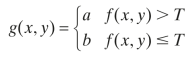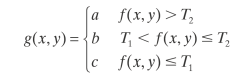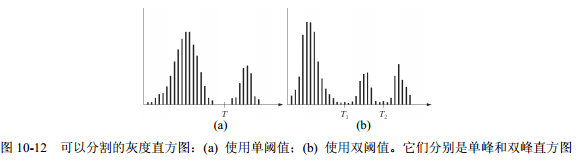## （2）基本全局阈值处理

1. 针对全局阈值选择初始估计值T；
2. 用T分割图像，G1是所有灰度值大于T的像素组成，G2是所有灰度值小于等于T的像素组成；
3. 分别计算G1和G2区域内的平均灰度值m1和m2；
4. 计算出新的阈值，取他们的m1和m2平均值数；
5. 重复步骤2.-4.，直到在连续的重复中，T的差异比预先设定的参数小为止；
6. 使用函数im2bw分割图像：g = im2bw(f,T/den)

f = imread('C:\Users\Public\Pictures\Sample Pictures\Fig0309(a).tif');
f = imnoise(f,'gaussian');
count = 0;
T = mean2(f);
done = false;
while ~done
count  = count+1;
g = f>T;
Tnext = 0.5*(mean(f(g))+mean(f(~g)));
done = abs(T-Tnext)<0.5;
T =Tnext;
end

count
T

g = im2bw(f,T/255);
subplot(131),imshow(f),title('带噪声的图像');
subplot(132),imhist(f),title('图像的直方图');
subplot(133),imshow(g),title('全局阈值分割的结果')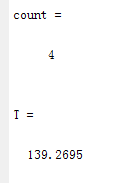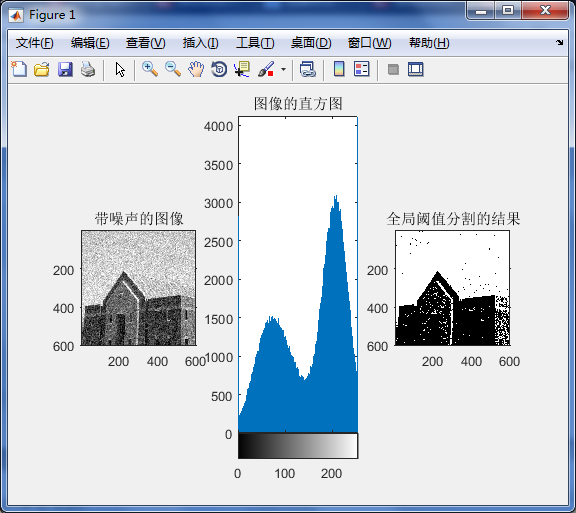## （3）使用Otsu’s方法的最佳全局阈值处理

Otsu’s方法的最佳方法是选择阈值k,最大类间方差σ2(k)来定义的：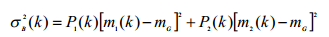[T,SM] = graythresh(f)   %SM是可分性度量,T是归一化的阈值


f = imread('C:\Users\Public\Pictures\Sample Pictures\Fig0309(a).tif');
f = imnoise(f,'gaussian');
[T,SM] = graythresh(f)
T*255


（基本全局算法）一般来说，高SM值说明灰度分成两类的可能性比较高。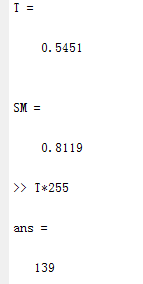f2 = imread('C:\Users\Public\Pictures\Sample Pictures\Fig1014(a).tif');
subplot(221),imshow(f2),title('原始图像');
subplot(222),imhist(f2),title('原始图像直方图');
count = 0;
T = mean2(f2);
done = false;
while ~done
count  = count+1;
g = f2>T;
Tnext = 0.5*(mean(f2(g))+mean(f2(~g)));
done = abs(T-Tnext)<0.5;
T =Tnext;
end
g = im2bw(f2,T/255);
subplot(223),imshow(g,[]),title('用基本全局算法分割结果')
[T,SM] = graythresh(f2);
SM
T*255
g = im2bw(f2,T);
subplot(224),imshow(g),title('用Otsu’s算法分割结果')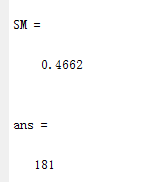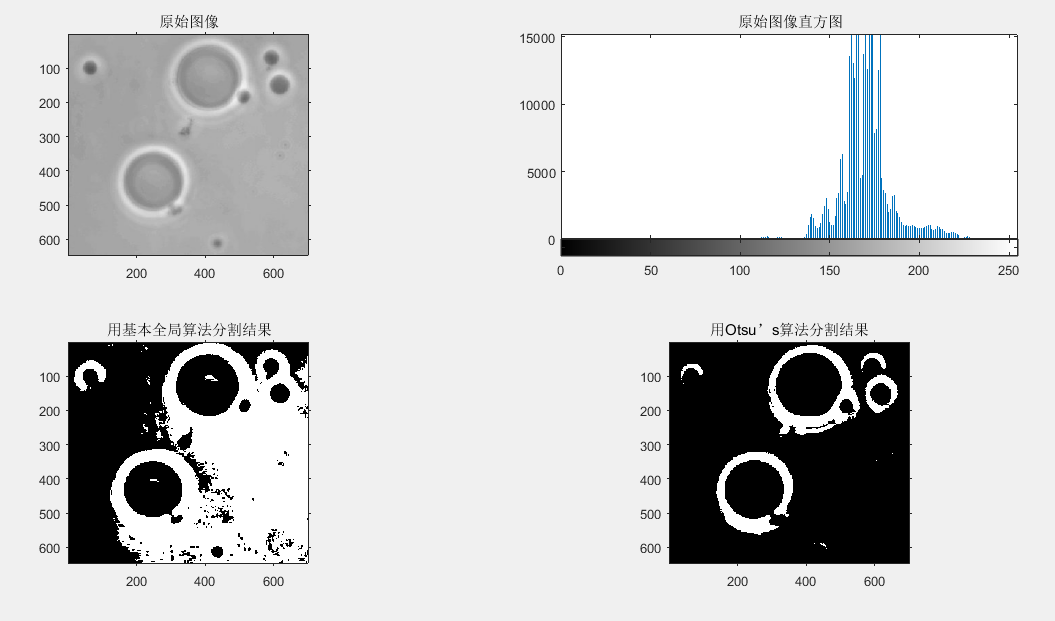实验分析： 可以看出，用基本全局阈值处理时细胞的分割效果并不是很好，没有能够分割清楚，是由于前景和背景的灰度级比较相近而不能够完全分离开，因此当使用了otsu’s算法进行分割时，可以完全将细胞（前景）和背景分割开来。尽管分离度的度量值比较低，但是还是可以准确地从背景中提取细胞。

## （4）使用图像平滑改进全局阈值处理

f = imread('C:\Users\Public\Pictures\Sample Pictures\Fig0513(a).tif');
subplot(241),imshow(f),title('原始图像');
fn = imnoise(f,'gaussian',0,0.038);
subplot(242),imshow(fn),title('具有高斯噪声的图像');
subplot(243),imhist(fn),title('具有高斯噪声的直方图');
Tn = graythresh(fn);
gn = im2bw(fn,Tn);
subplot(244),imshow(gn),title('用otsu’s分割的图像');
w = fspecial('average',5);
fa = imfilter(fn,w,'replicate');
subplot(245),imshow(fa),title('平滑后的图像');
subplot(246),imhist(fa),title('平滑后的直方图');
Ta = graythresh(fa);
ga =im2bw(fa,Ta);
subplot(247),imshow(ga),title('平滑后并用otsu’s分割的图像');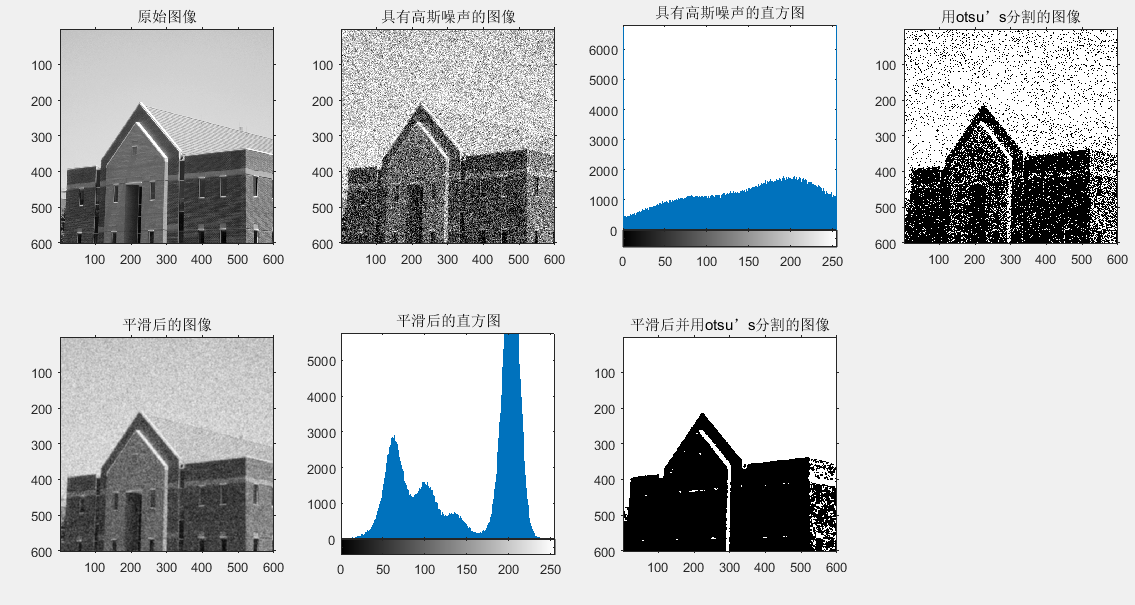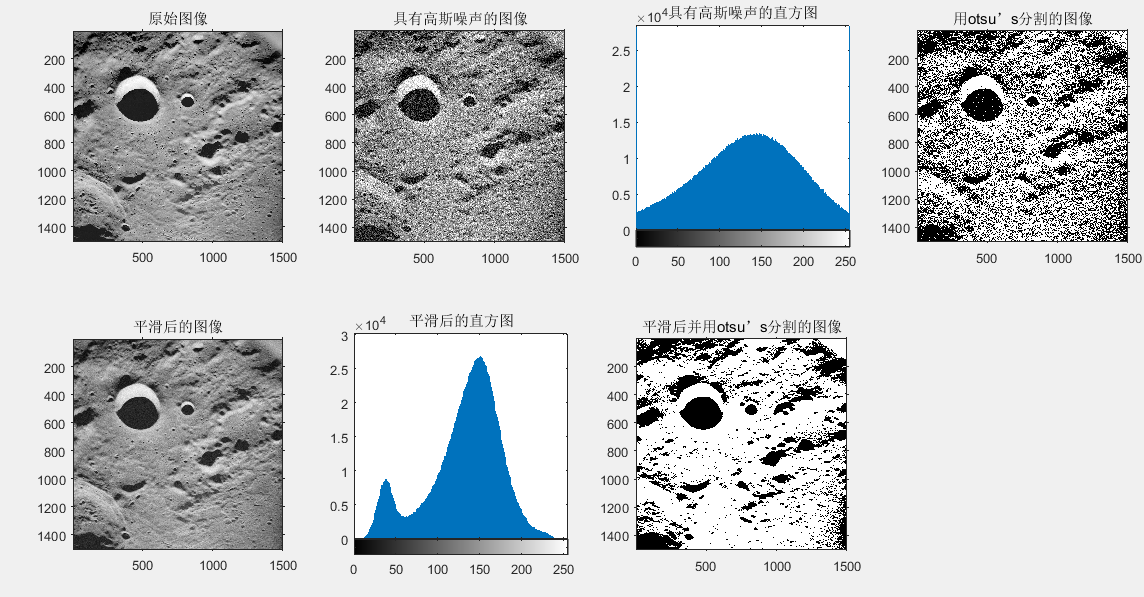实验分析： 当加入高斯噪声的图像，所显示的直方图并不能使用阈值处理进行分割图像，所以经过otsu’s全局阈值处理操作后发现，原始图像和噪声并不能够分离开。当使用平滑滤波器进行图像改善之后，从其直方图看出，可以使用阈值处理进行分割图像了，所以最后的效果图显示，图像与噪声分割开来，因此可以使用otsu’s全局阈值处理对图像进行操作。

## （5）使用边缘改进全局阈值处理

1. 用一种边缘查找方式计算图像的模板的值。
2. 通过百分比指定阈值。由于计算的边缘模板值中有很多噪声，所以可以将计算值排序，并选择百分比相对高的值(大于百分下的值的阈值)作为阈值。
3. 根据指定的阈值，对第一步的图像边缘的值进行选择。使高于阈值的像素点值为1，低于的值为零，由此选择出部分边缘点的二值图像(模板)。
4. 用刚才计算出来的模板与原图像相乘，获得一幅新的图像。
5. 对新的图像使用otsu进行分割。

I = percentile2i(h,p)


f = tofloat(imread('C:\Users\Public\Pictures\Sample Pictures\Fig1013(a).tif'));
subplot(231),imshow(f),title('原图像');
subplot(232),imhist(f),title('原图像直方图');
sx = fspecial('sobel');
sy = sx';
gx = imfilter(f,sx,'replicate');
gy = imfilter(f,sy,'replicate');

Q = percentile2i(h,0.999);

subplot(233),imshow(markerImage),title('以99.9%进行阈值处理后的梯度幅值图像');
fp = f.*markerImage;
subplot(234),imshow(fp),title('原图像与梯度幅值乘积的图像');
hp = imhist(fp);
hp(1) = 0;
subplot(235),bar(hp),title('原图像与梯度幅值乘积的直方图');
T = otsuthresh(hp);
T*(numel(hp)-1)
g = im2bw(f,T);
subplot(236),imshow(g),title('改进边缘后的图像')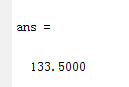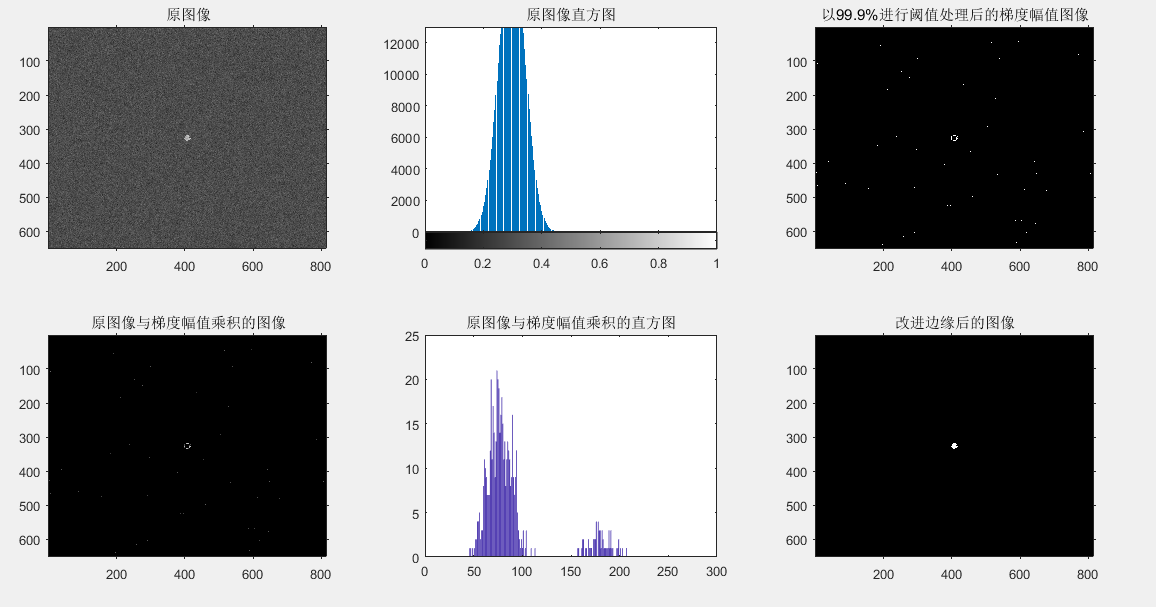f = tofloat(imread('C:\Users\Public\Pictures\Sample Pictures\Fig1017(a).tif'));
subplot(231),imshow(f),title('原始图像');
subplot(232),imhist(f),title('原始图像的直方图');
hf = imhist(f);
[Tf SMf] = graythresh(f);
gf = im2bw(f,Tf);
subplot(233),imshow(gf),title('对原始图像进行分割的图像');
w= [-1 -1 -1;-1 8 -1;-1 -1 -1];
lap = abs(imfilter(f,w,'replicate'));
lap = lap/max(lap(:));
h = imhist(lap);
Q = percentile2i(h,0.995);
markerImage = lap >Q;
fp = f.*markerImage;
subplot(234),imshow(fp),title('标记图像与原图像的乘积');
hp = imhist(fp);
hp(1) =0;
subplot(235),bar(hp)
T = otsuthresh(hp);
g = im2bw(f,T);
subplot(236),imshow(g),title('修改边缘后的阈值处理图像')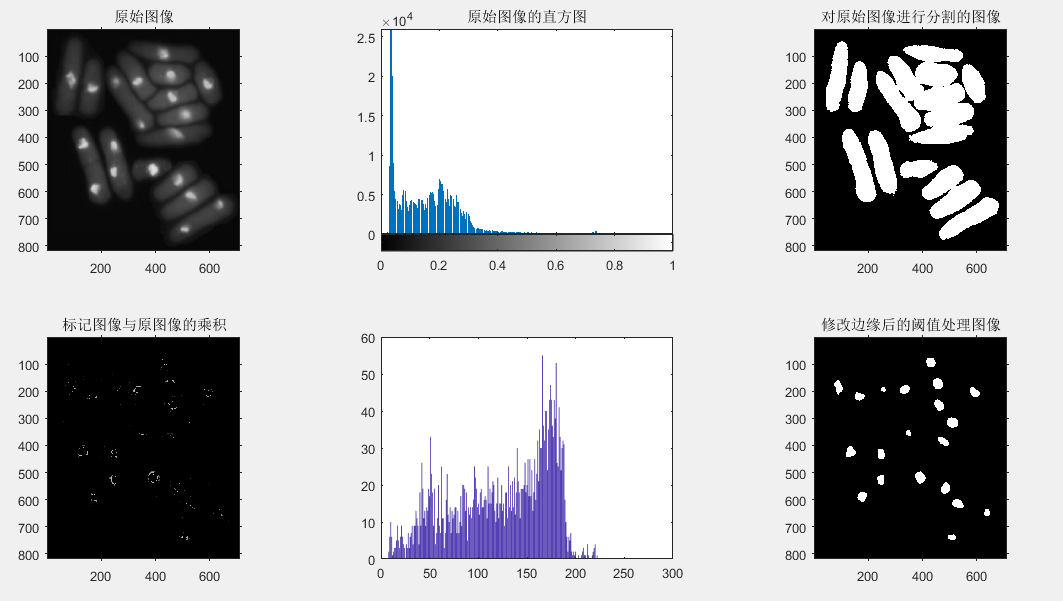实验分析： 在之前的学习知道，拉普拉斯算法是用于图像的锐化，把图像的边缘提取出来。在这个部分中，用拉普拉斯边缘信息改进全局阈值处理也是一样的，首先将原始图像的边缘提取出来，然后将原始图像与已标记好的图像进行相乘，得到的就是实验中所需要的图像，最终图像就如边缘改进结果那样，修改边缘信息提取出来，再用阈值进行处理。

## （6）基于局部统计的可变阈值处理

function mean = localmean(f,nhood)
if nargin ==1
nhood = ones(3)/9;
else
nhood = nhood/sum(nhood(:));
end
mean = imfilter(tofloat(f),nhood,'replicate');


function g = localthresh(f,nhood,a,b,meantype)
f = tofloat(f);
SIG = stdfilt(f,nhood);
if nargin == 5 && strcmp(meantype,'global')
MEAN = mean2(f);
else
MEAN = localmean(f,nhood);
end
g = (f > a*SIG) & (f > b*MEAN);


[TGlobal] = graythresh(f);
gGlobal = im2bw(f,TGlobal);
subplot(131),imshow(gGlobal),title('用otsu方法分割的图像');
g = localthresh(f,ones(3),30,1.5,'global');
SIG = stdfilt(f,ones(3));
subplot(132),imshow(SIG,[]),title('局部标准差图像');
subplot(133),imshow(g),title('局部阈值处理后的图像');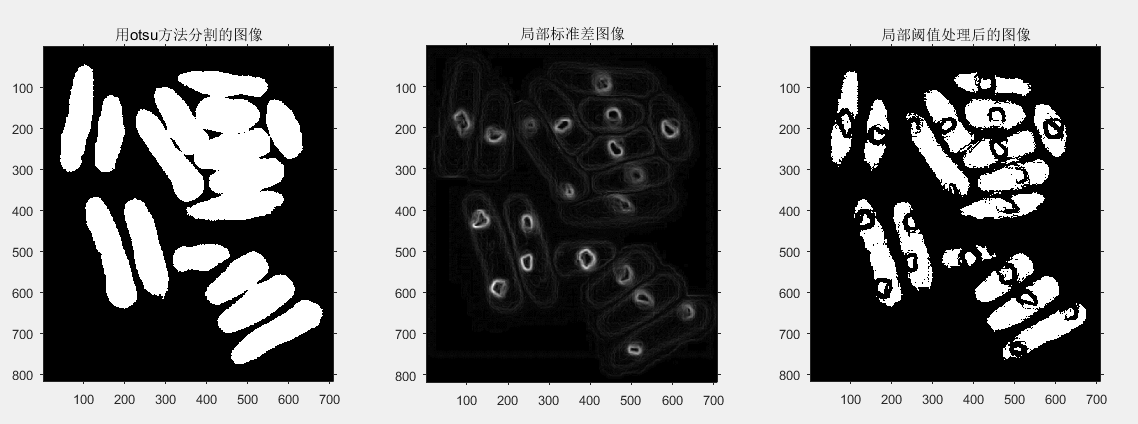实验分析： 观察原图像发现，图像可以分为三种灰度级，发现除了细胞核的部分，细胞核与细胞的灰度级差别并不是很大，因此当我们使用全局阈值处理时，不能将里面的细胞核与细胞分离开，得到的阈值处理如otsu方法分割图像所示。当实验使用局部可变的阈值处理时，计算出图像的局部标准差，可以将细胞与细胞核分离开，因此当再次使用阈值处理时可以完整的将细胞与细胞核分离开，获得最终的处理结果。

## （7）使用移动平均的图像阈值处理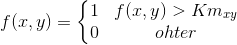function g = movingthresh(f, n, K)
f = tofloat(f);
[M, N] = size(f);
if (n < 1) || (rem(n, 1) ~= 0)
error('n must be an integer >= 1.')
end
if K < 0 || K > 1
error('K must be a fraction in the range [0, 1].')
end
f(2:2:end, :) = fliplr(f(2:2:end, :));
f = f'; 	% Still a matrix.
f = f(:)'; % Convert to row vector for use in function filter.
maf = ones(1, n)/n; 	% The 1-D moving average filter.
ma = filter(maf, 1, f); % Computation of moving average.
g = f > K * ma;
g = reshape(g, N, M)';
g(2:2:end, :) = fliplr(g(2:2:end, :));


f = imread('C:\Users\Public\Pictures\Sample Pictures\Fig1019(a).tif');
subplot(131),imshow(f),title('原始图像');
T =graythresh(f);
g1 = im2bw(f,T);
subplot(132),imshow(g1),title('用otsu全局阈值分割后的图像');
g2 = movingthresh(f,20,0.5);
subplot(133),imshow(g2),title('用移动平均局部阈值分割后的图像');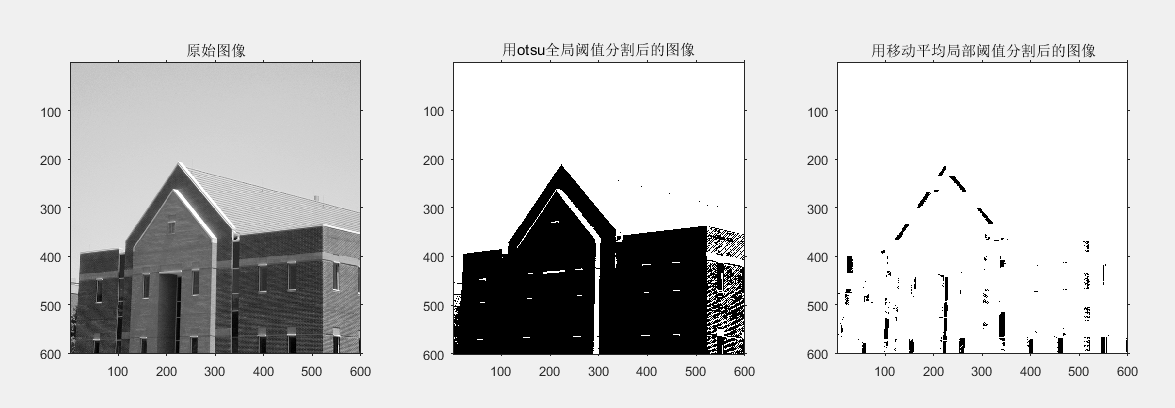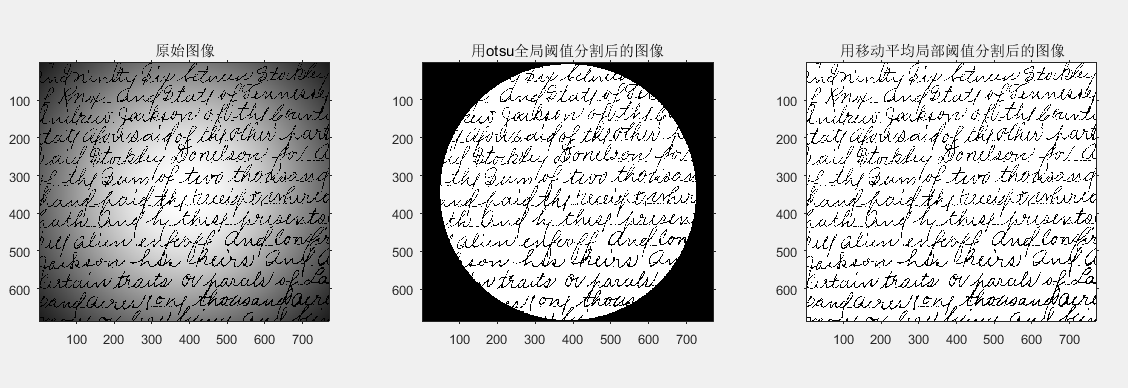实验分析： 实验之前就说过，移动平均的图像阈值处理适用于处理打印图像和手写文本图像这种类型。对比房子的移动平均处理看出，最后所获得的图像不是实验所需要的图像，因此这种方法并不适用于这种图像处理。在处理手写文本图像的时候，经过局部移动平均阈值处理后，所获得的结正是我们所需要的。

# （四）基于区域的分割

## （1）基本表达式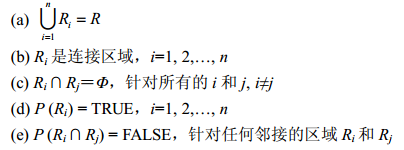## （2）区域生长

（1）表示区域的初始化种子的选择：在区域生长过程中，这些不同区域点合适属性的选择。
（2）基于图像具体属性的像素生长不一定是好的分割。在区域生长过程中，不应该使用连通性或邻接信息。
（3）相似性：相似性表示在灰度级中观察在两个空间邻接像素之间或像素集合的平均灰度级间的最小差分，它们将产生不同的区域。如果这个差分比相似度阈值小，则像素属于相同的区域。
（4）区域面积：最小面积阈值与像素中的最小区域大小有关。在分割的图像中，没有区域比这个阈值小，它由用户定义。（来自百度百科）

[g,NR,SI,TI] = regiongrow(f,S,T)  %f是被分割图像，S/T可以是数组或标量，范围是[0,1]内


f = imread('C:\Users\Public\Pictures\Sample Pictures\Fig0309(a).tif');
subplot(221),imshow(f),title('原始图像');
[g,NR,SI,TI]=regiongrow(f,255,65);%种子的像素值为255，65为阈值
subplot(222),imshow(SI),title('种子点的图像');
subplot(223),imshow(TI),title('所有通过阈值测试的像素');
subplot(224),imshow(g),title('对种子点进行8连通分析后的结果');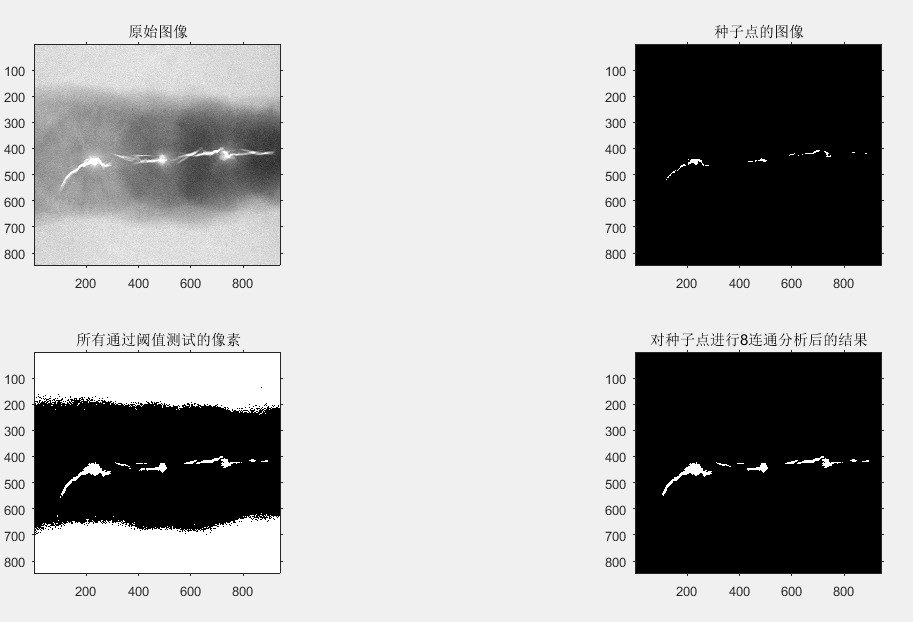## （3）区域分离和聚合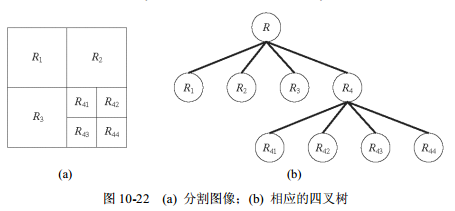z = qtdecomp(f,@split_test,parameters)  %split_test用来决定某个区域是否进行分离


[vals,r,c] = qtgetblk(f,z,m)  %vals是数组，r和c事行列坐标的向量，z是返回的稀疏矩阵


g = splitmerge(f,mindim,@predicate)  %mindim是分解中允许的最小块


predicate函数是用户自定义的，用于考察该区域的是否符合要求

flag = predicate(region)


f = imread('C:\Users\Public\Pictures\Sample Pictures\Fig1023(a).tif');
subplot(231),imshow(f),title('原始图像');
g32 = splitmerge(f,32,@predicate) %使用最小块为32进行分割
subplot(232),imshow(g32),title('使用最小块为32进行分割图像');
g16 = splitmerge(f,16,@predicate) %使用最小块为16进行分割
subplot(233),imshow(g16),title('使用最小块为16进行分割图像');
g8= splitmerge(f,8,@predicate) %使用最小块为8进行分割
subplot(234),imshow(g8),title('使用最小块为8进行分割图像');
g4 = splitmerge(f,4,@predicate) %使用最小块为4进行分割
subplot(235),imshow(g4),title('使用最小块为4进行分割图像');
g2 = splitmerge(f,2,@predicate) %使用最小块为2进行分割
subplot(236),imshow(g2),title('使用最小块为2进行分割图像');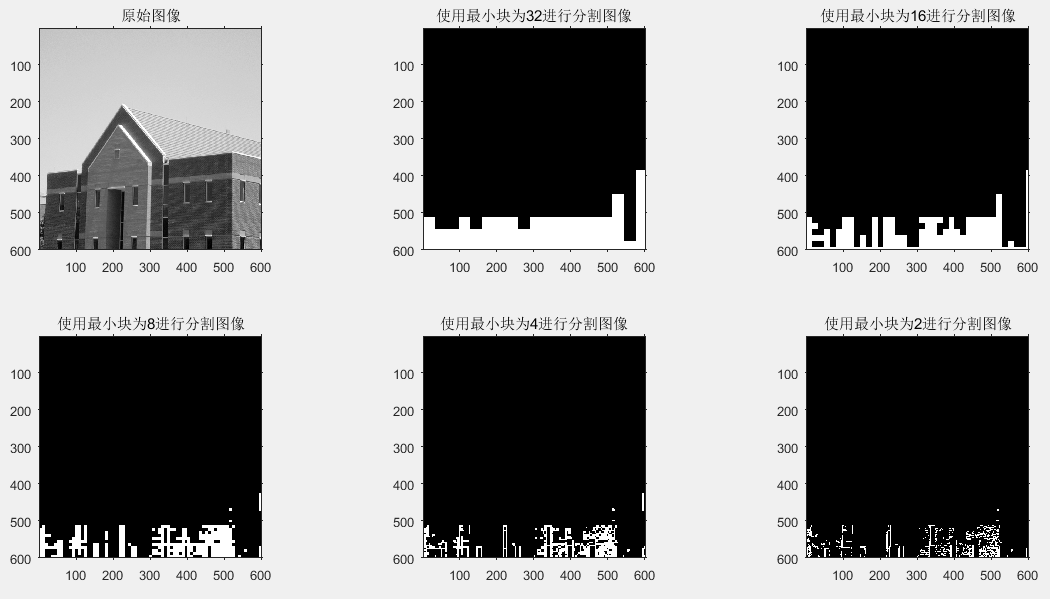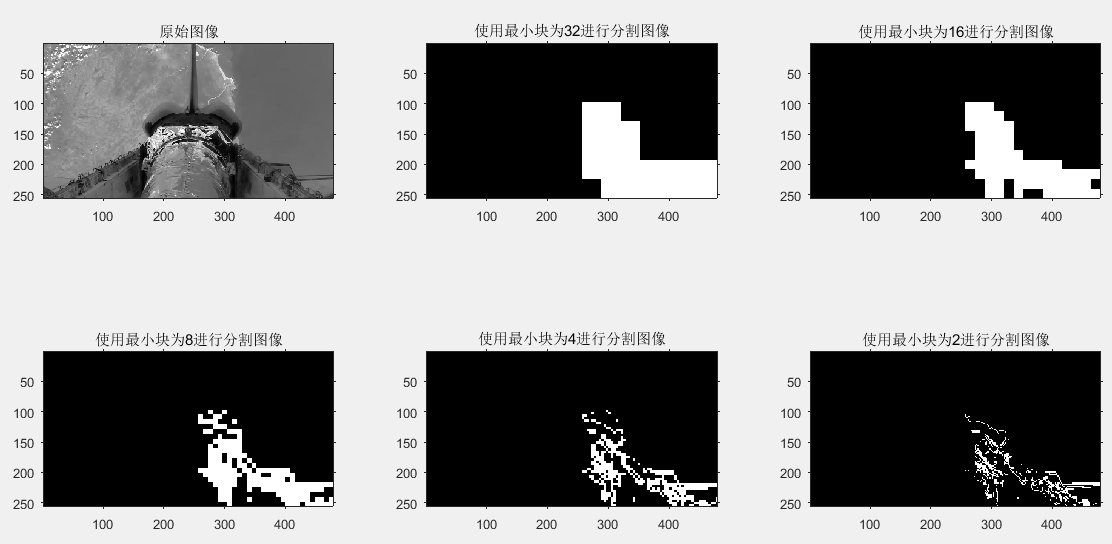# （五）使用分水岭变换的分割

## （1）使用距离变换的分水岭分割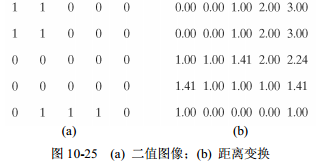f = imread('C:\Users\Public\Pictures\Sample Pictures\Fig0513(a).tif');
subplot(231),imshow(f),title('原始图像');
g = im2bw(f,graythresh(f));   %把图像变换成二值图像
subplot(232),imshow(g),title('二值图像');
gc = ~g;
subplot(233),imshow(gc),title('补的图像');
D = bwdist(gc);
subplot(234),imshow(D),title('距离变换图像');
L = watershed(-D);
w = L == 0;
subplot(235),imshow(w),title('负分水岭脊的图像');
g2 = g&-w;
subplot(236),imshow(g2),title('黑色重叠后的图像');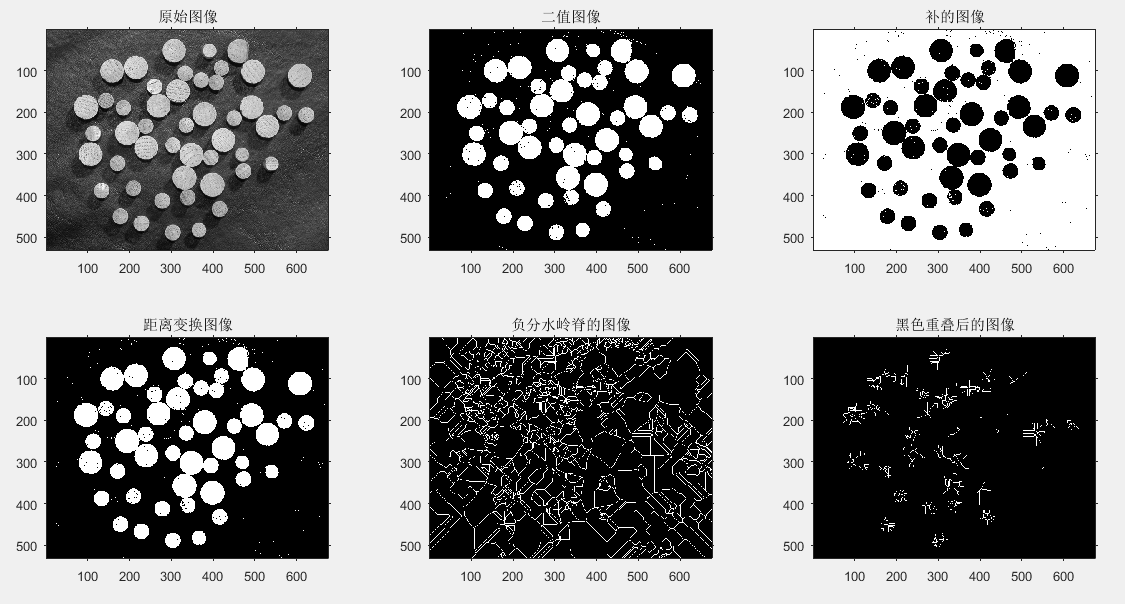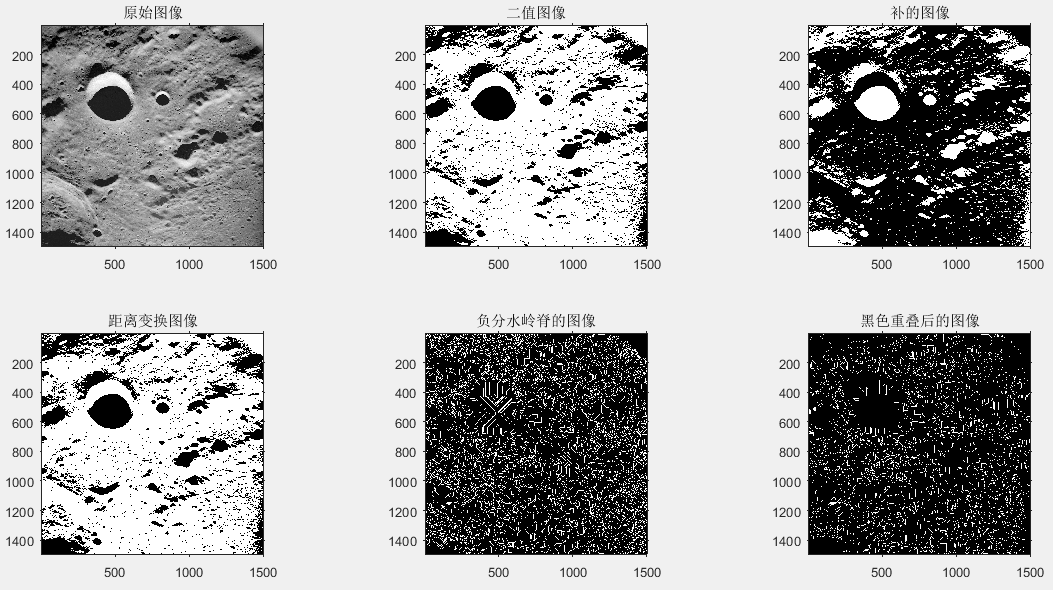## （2）使用梯度的分水岭分割

f = imread('C:\Users\Public\Pictures\Sample Pictures\Fig1014(a).tif');
subplot(221),imshow(f),title('原始图像');
h = fspecial('sobel');
fd = tofloat(f);
g = sqrt(imfilter(fd,h,'replicate').^2+imfilter(fd,h,'replicate').^2);
subplot(222),imshow(g,[]),title('梯度和分水岭分割幅度图像');
L =watershed(g);
wr = L == 0;
subplot(223),imshow(wr),title('严重分割过分割后图像');
g2 = imclose(imopen(g,ones(3,3)),ones(3,3));
L2 = watershed(g2);
wr2 = L2 == 0;
f2 = f;
f2(wr2) = 255;
subplot(224),imshow(f2),title('平滑梯度图像后的分水岭变换');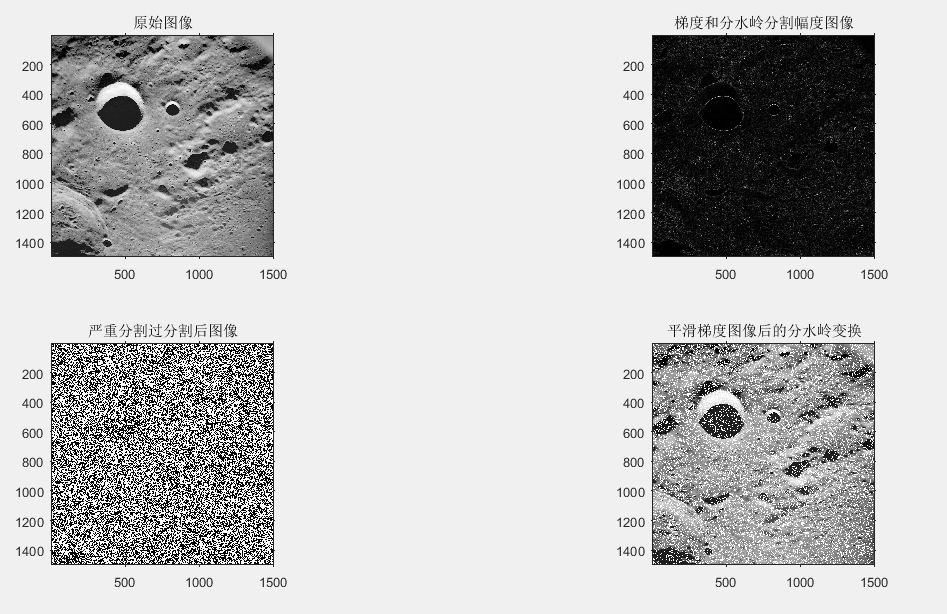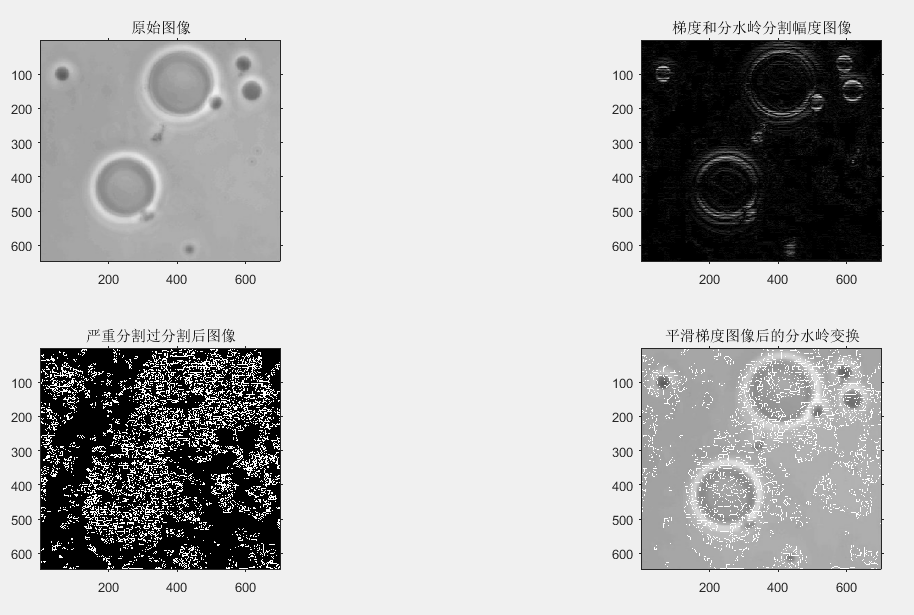实验分析： 观察两组图像，当梯度的分水岭分割时，所获得的图像基本上满足实验的效果，但由于严重的过度分割后，图像已不再是需要得到的效果，使得图像分割没有意义了。使用平滑梯度图像的分水岭分割后，虽然产生了一些缺陷（图像上被一些类似噪声污染），但没有影响图像的分割效果。基于梯度的分水岭分割法是修改梯度函数使得集水盆只响应想要探测的目标，再对梯度图像进行阈值处理，以消除灰度的微小变化产生的过度分割。

## （3）控制标记符的分水岭分割

f = imread('C:\Users\Public\Pictures\Sample Pictures\Fig1028(a).tif');
subplot(241),imshow(f),title('原始图像');
h = fspecial('sobel');
fd = tofloat(f);
g = sqrt(imfilter(fd,h,'replicate').^2+imfilter(fd,h,'replicate').^2);
L =watershed(g);
wr = L == 0;
subplot(242),imshow(wr),title('梯度幅度图像进行分水岭变换图像');
rm = imregionalmin(g);  %计算图像中所有的局部小区域的位置
subplot(243),imshow(rm),title('梯度幅值的局部小区域图像');
im = imextendedmin(f,2);  %用于计算比临近点更暗的图像中“低点”的集合
fim = f;
fim(im) = 175;
subplot(244),imshow(f,[]),title('内部标记符图像');
Lim = watershed(bwdist(im));
em = Lim == 0;
subplot(245),imshow(em,[]),title('外部标记符图像');
g2 = imimposemin(g,im|em);  %用来修改一幅图像,使得其只在指定的位置处取得局部最小值
subplot(246),imshow(g2),title('修改后的梯度幅值图像');
L2 = watershed(g2);
f2 = f;
f2(L2 == 0) = 255;
subplot(247),imshow(f2),title('最后的分割图像');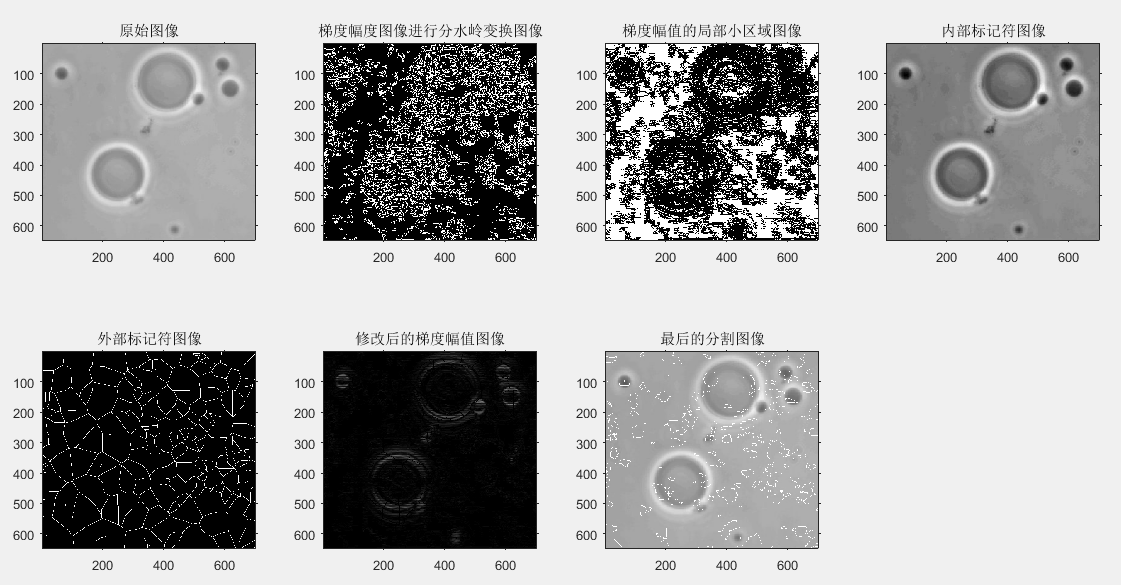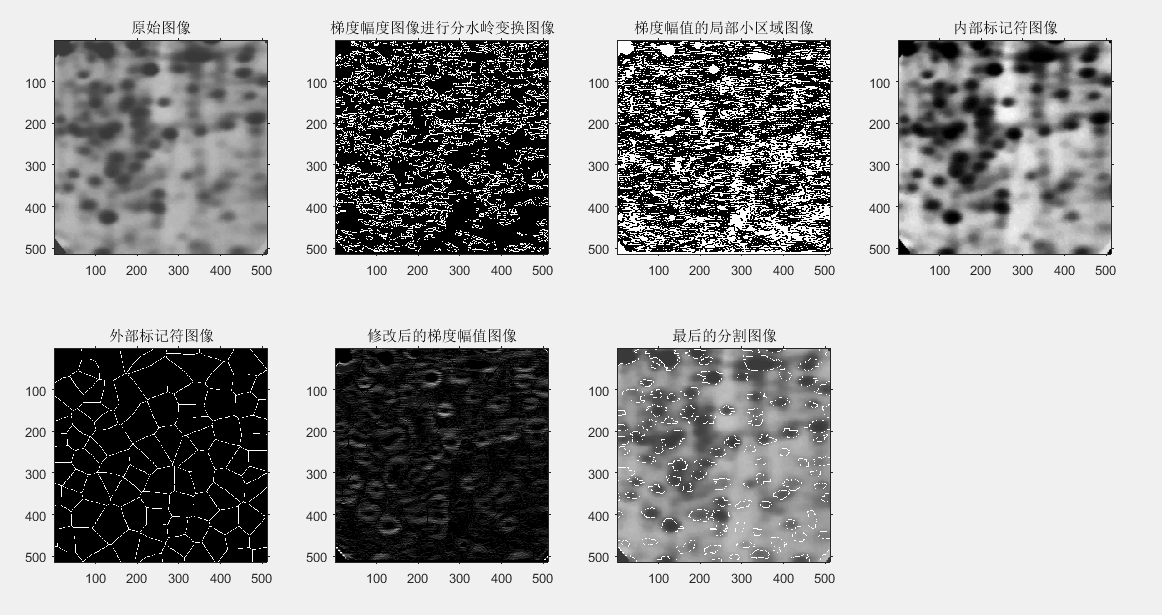实验分析： 当直接使用梯度幅度方法进行分割处理时，发现图像过度分割非常严重，而这样的分割是没有意义的，并不是我们所需要的。当采用控制标记符分水岭分割算法时，能调整过度分割的界限，设置内部标记符和外部标记符，把所需要的内外部标记符联合起来，再次进行分割，因此可以得到我们所需要的分割效果。

## 图像分割：2.基于边缘检测的图像分割方法

2018-08-12 22:36:03 ml_ai_sun 阅读数 15302
• ###### matlab边缘检测和图像分割

matlab边缘检测 <img src="https://img-bss.csdn.net/201903190616489027.jpg" alt="" /></p>

7课时 101分钟 981人学习 刘昱显
免费试看

### 二、微分算子

常用的一阶微分算子有Roberts、Prewitt、Sobel等算子，常用的二阶微分算子有Laplace和Kirsh等算子。在实际处理操作中常用模板矩阵与图像像素值矩阵卷积来实现微分运算。

由于垂直边缘的方向上的像素点和噪声都是灰度不连续点，所以变换到频域时，在频域均为高频分量，直接采用微分运算不可避免地会受到噪声的很大影响。 因此，微分算子只适用于图像噪声比较少的简单图像。针对此问题，LoG算子（Laplace of Gaussian）和Canny算子采取的方法是，先对图像进行平滑滤波，然后再用微分算子与图像进行卷积操作，这样处理会得到比较好的边缘检测结果。其中，LoG算子是采用Laplacian算子计算高斯函数的二阶导数，Canny算子是高斯函数的一阶导数，两种算子在抑制噪声和检测边缘之间取得了比较好的平衡。

1、roberts算子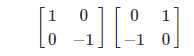2、prewitt算子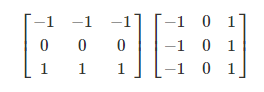3、sobel算子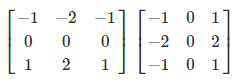4、LoG算子（二阶微分算子）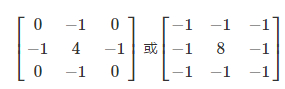LoG算子的特点：
1. 图像与高斯滤波器进行卷积，既平滑了图像又降低了噪声，孤立的噪声点和较小的结构组织将被滤除。
2. 在边缘检测时则仅考虑那些具有局部梯度最大值的点为边缘点，用拉普拉斯算子将边缘点转换成零交叉点，通过零交叉点的检测来实现边缘检测。
3. 缺点是在过滤噪声的同时使得原有的边缘一定程度上被平滑了。

5、canny算子

Canny算子检测边缘点的方法基本思想是寻找图像梯度的局部最大值。
评价一个边缘检测算子的，一般考虑如下三个指标：
1. 低失误概率：在尽可能把所有边缘检测到的同时，减少将非边缘误判为边缘；
2. 高位置精度：检测出的边缘是真正的边界，检测到的边缘位置足够精确；
3. 检测得到的边界是单像素宽。
针对这三个指标，Canny在设计检测算子时提出了边缘检测算子的三个准则：
1. 信噪比准则；
2. 定义精度准则；
3. 单边缘响应准则。

遵循这三个准则，Canny算子设计实现的步骤如下：
（1）首先用高斯滤波模板进行卷积以平滑图像；
（2）利用微分算子，计算梯度的幅值和方向；
（3）对梯度幅值进行非极大值抑制。即遍历图像，若某个像素的灰度值与其梯度方向上前后两个像素的灰度值相比不是最大，那么这个像素值置为0，即不是边缘；
（4）使用双阈值算法检测和连接边缘。即使用累计直方图计算两个阈值，凡是大于高阈值的一定是边缘；凡是小于低阈值的一定不是边缘。如果检测结果大于低阈值但又小于高阈值，那就要看这个像素的邻接像素中有没有超过高阈值的边缘像素，如果有，则该像素就是边缘，否则就不是边缘。